# MSBSHSE Solutions For Class 9 Maths Part 1 Chapter 1 Sets

MSBSHSE Solutions For Class 9 Maths Part 1 Chapter 1 Sets are provided here for students to practice and prepare for their exam. All these solutions have stepwise explanations, which aim at a student’s better understanding. The MSBSHSE solutions available at BYJU’S for Sets will help the students to clear their doubts and to get full marks for the questions from this chapter, in their final exams. Students can easily master the concepts of Sets. We come across Types of sets, Venn Diagrams, intersection and union of sets in this chapter. These solutions are really helpful to get a better understanding of concepts of sets. Maharashtra State Board Solutions Class 9 can be downloaded in PDF format. These solutions help to develop confidence among students who are preparing for exams.

## Download the PDF of Maharashtra Solutions For Class 9 Maths Part 1 Chapter 1 Sets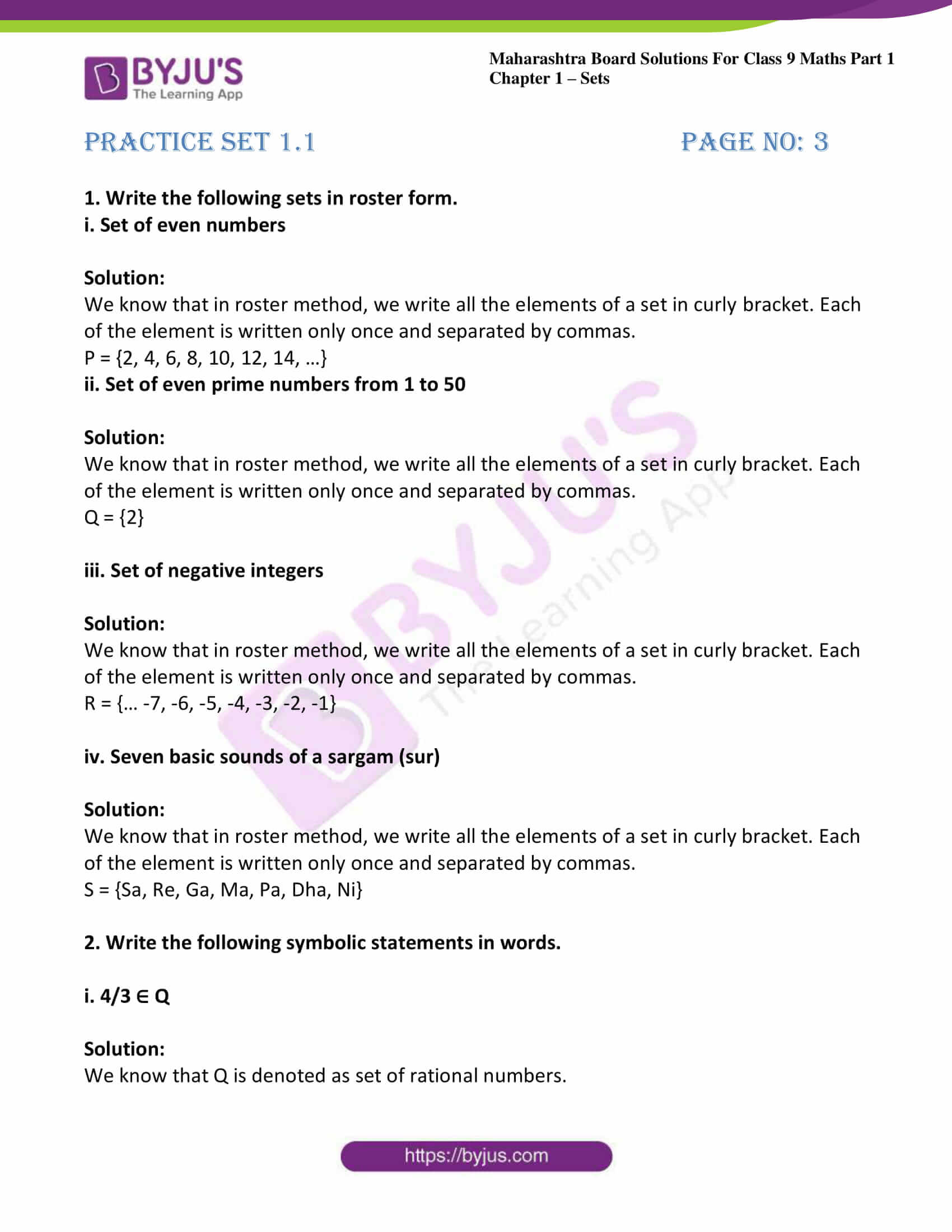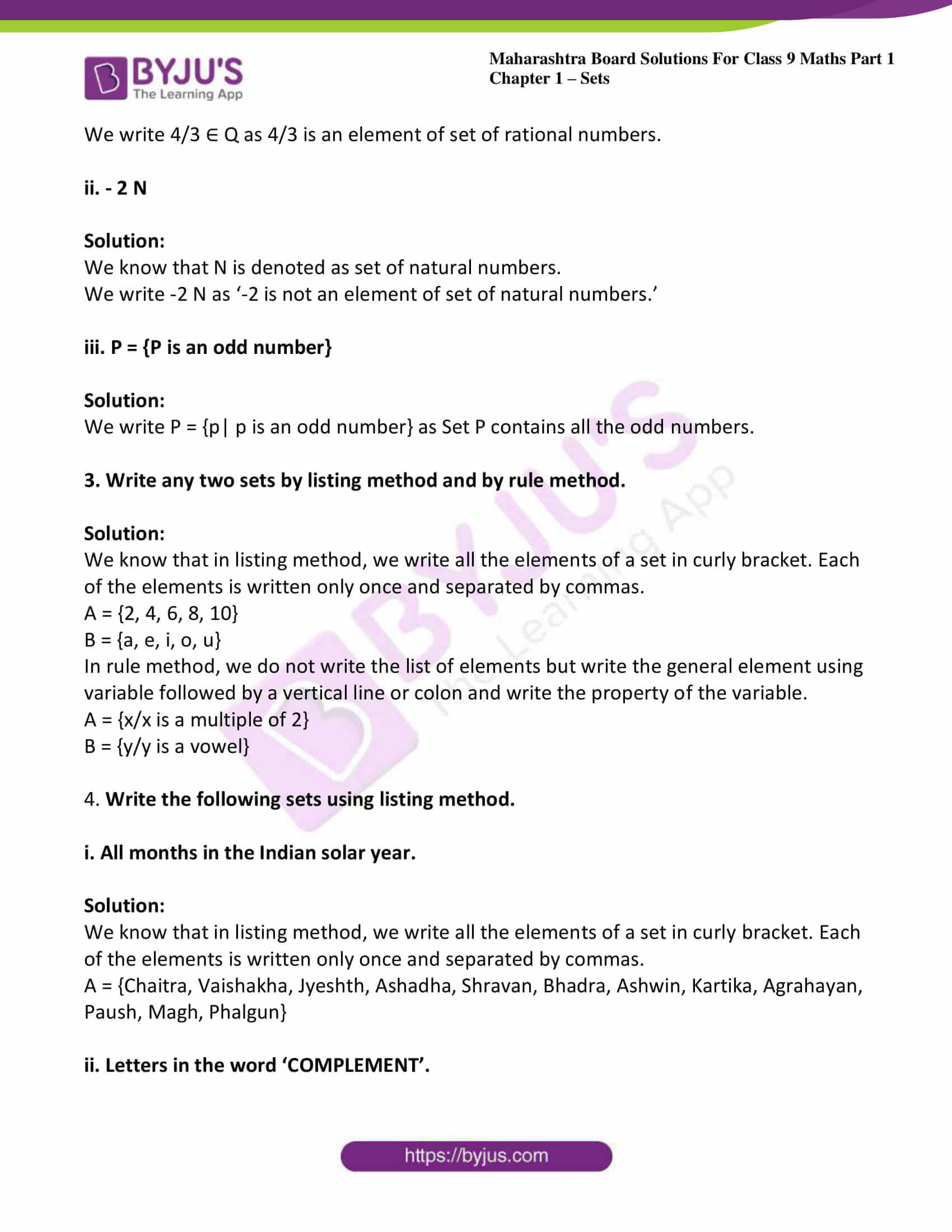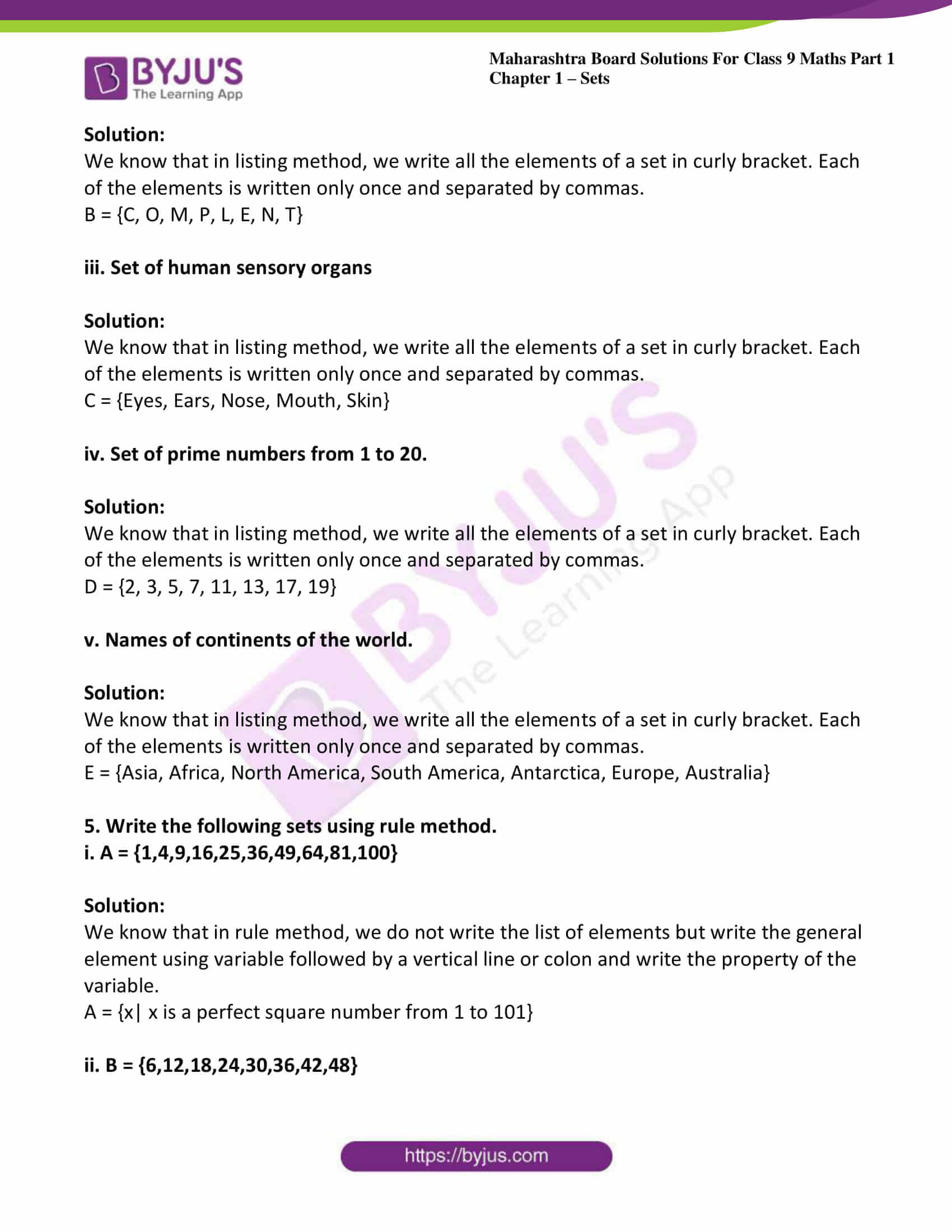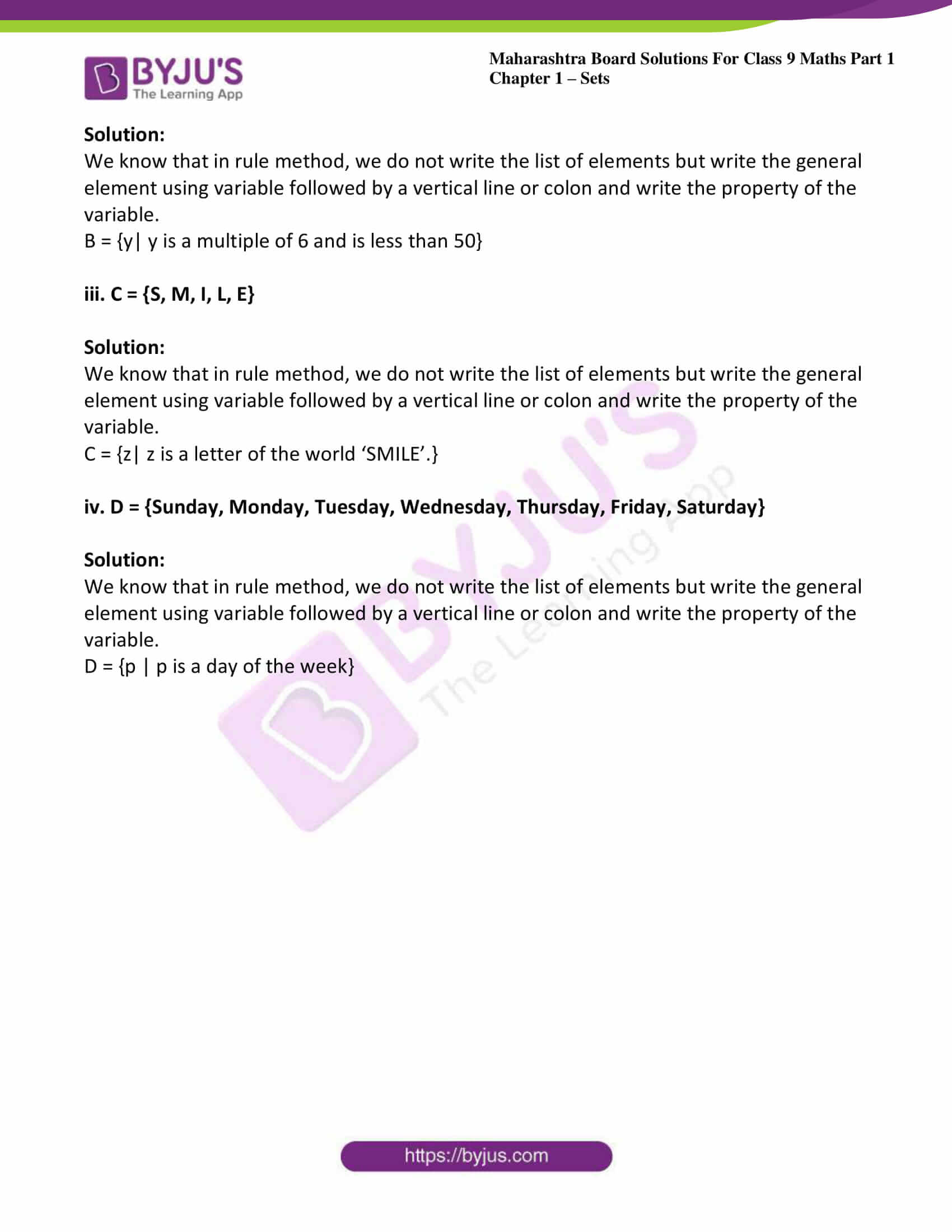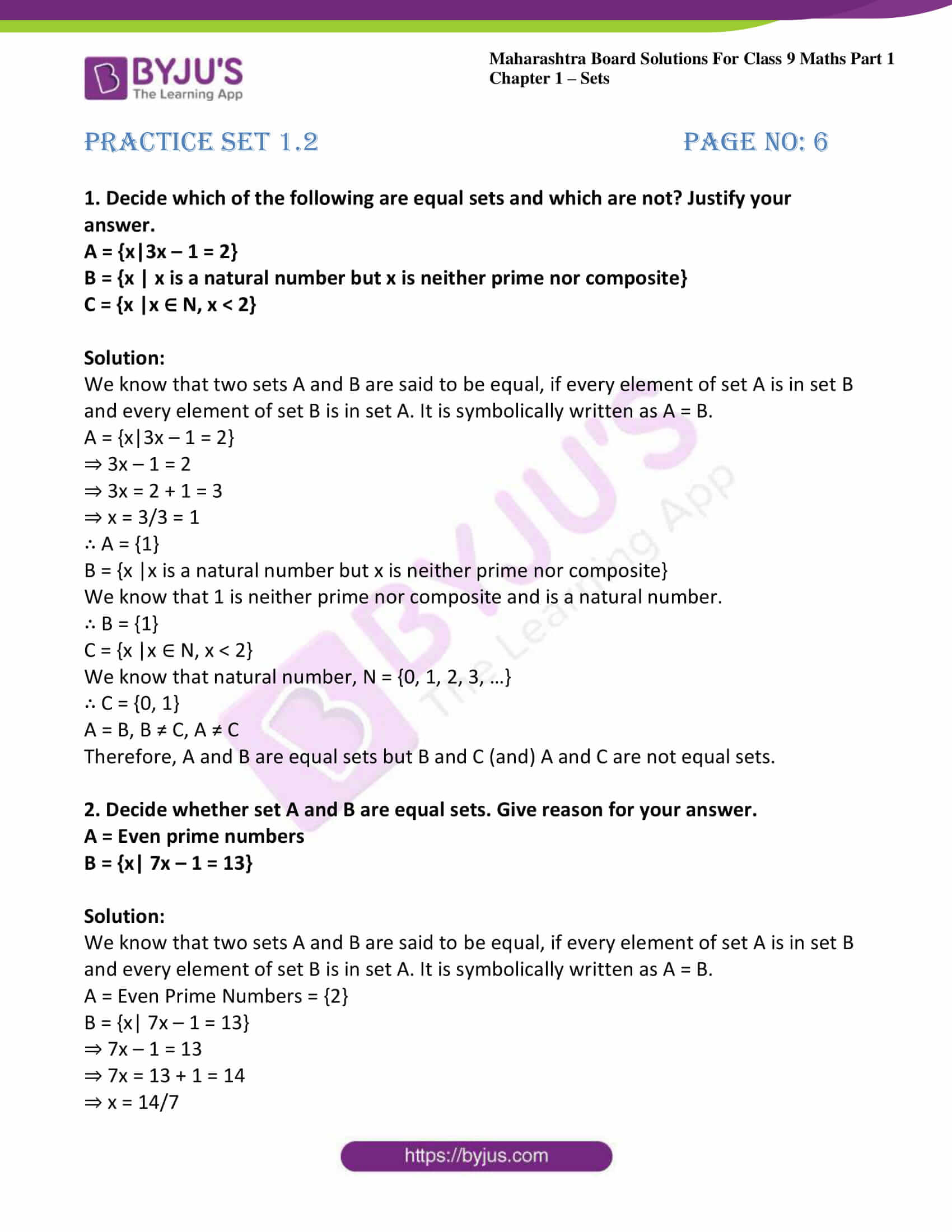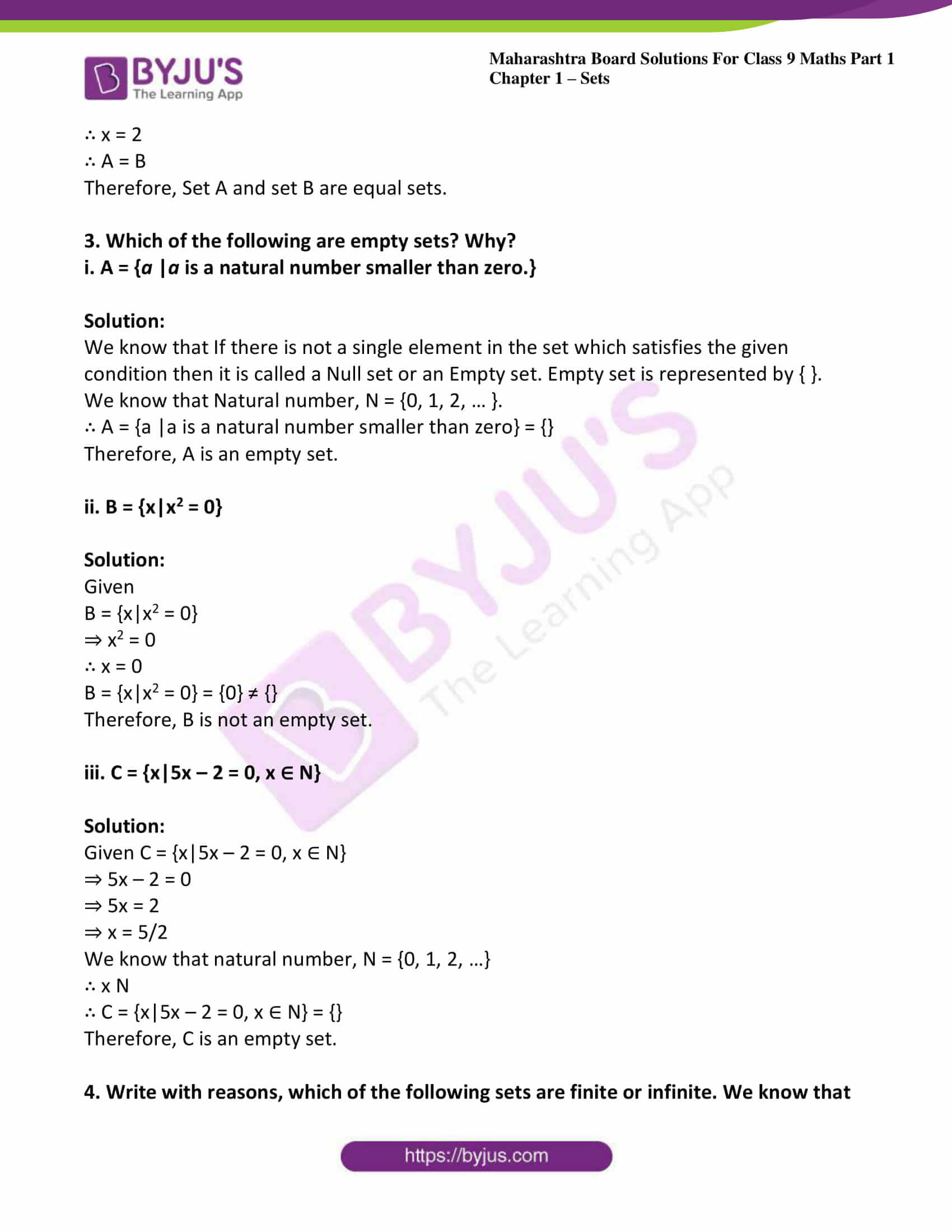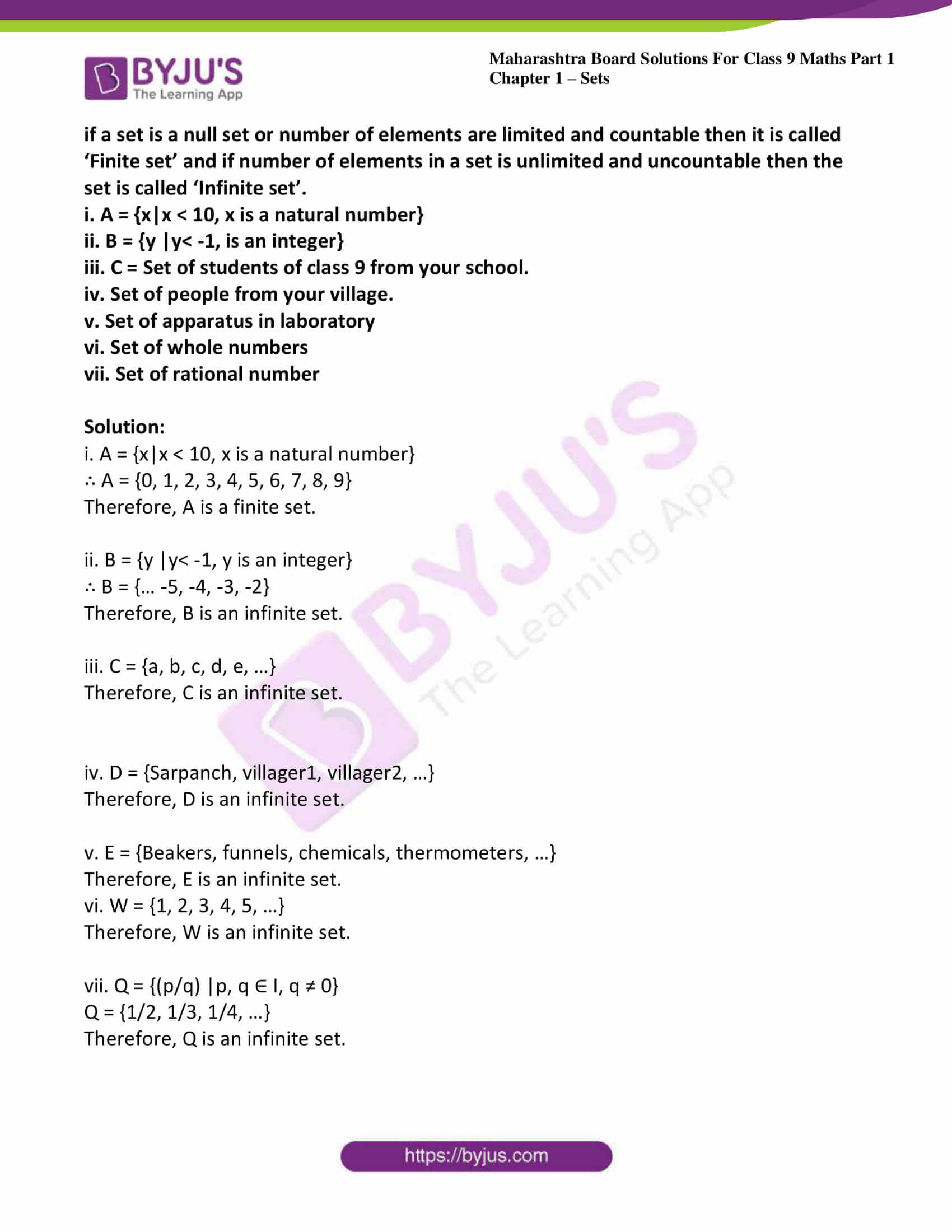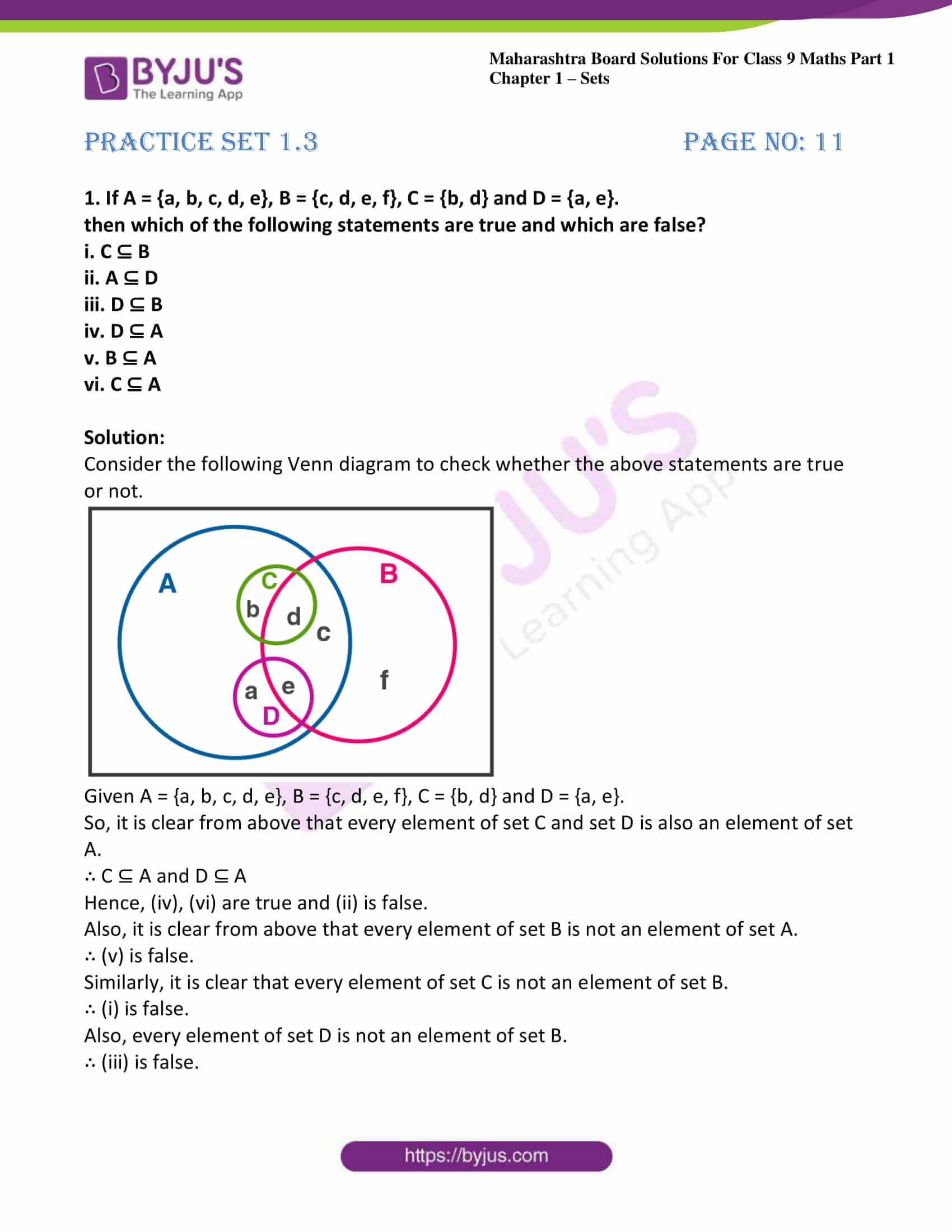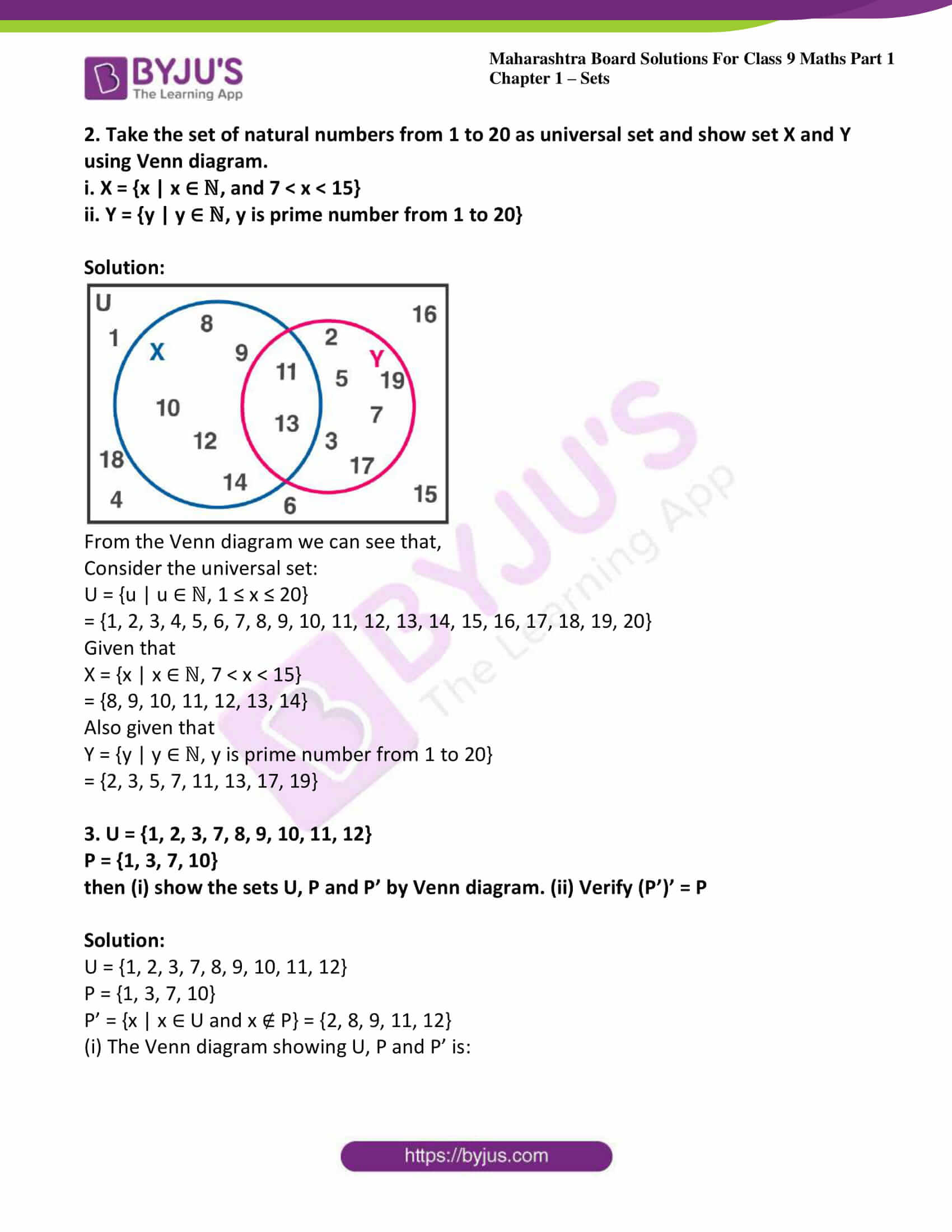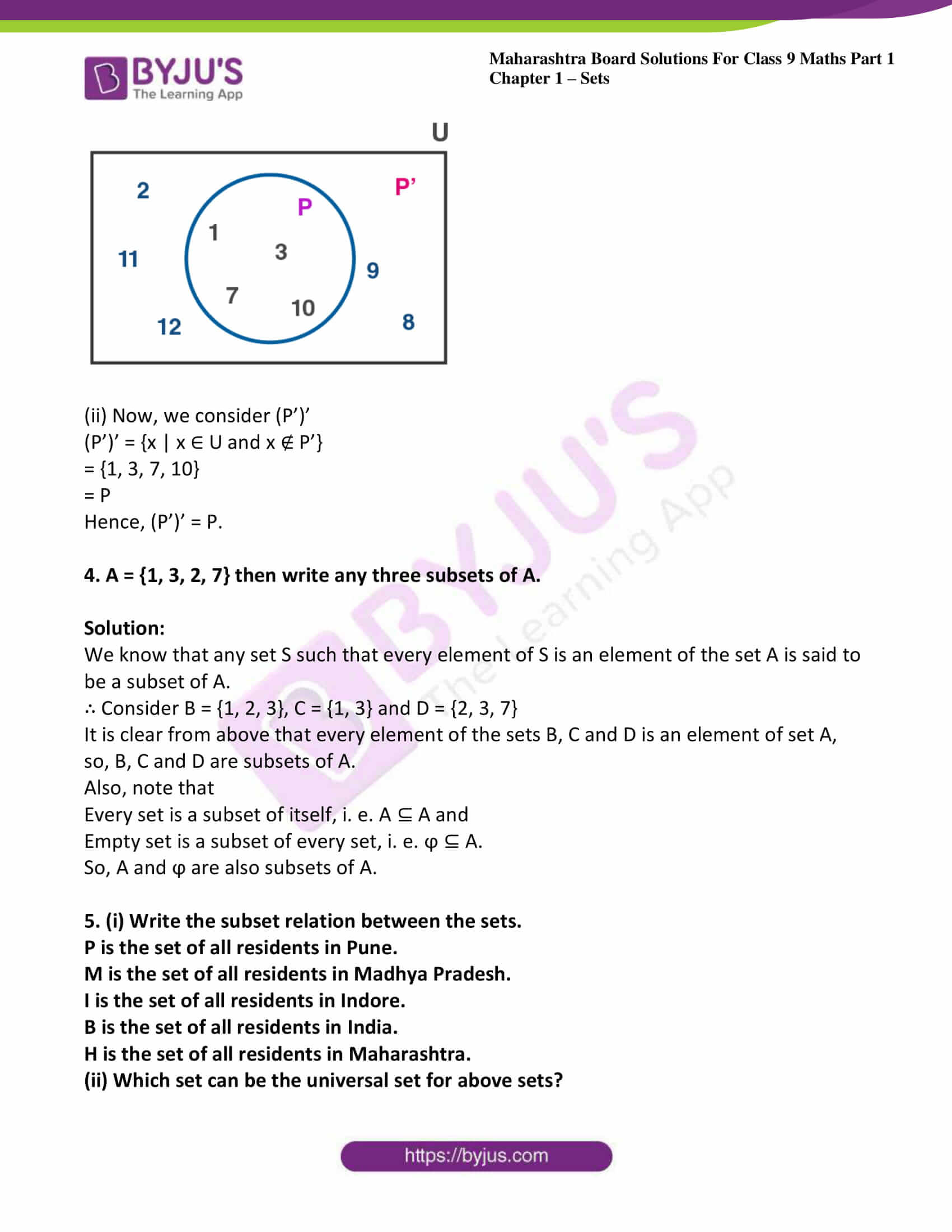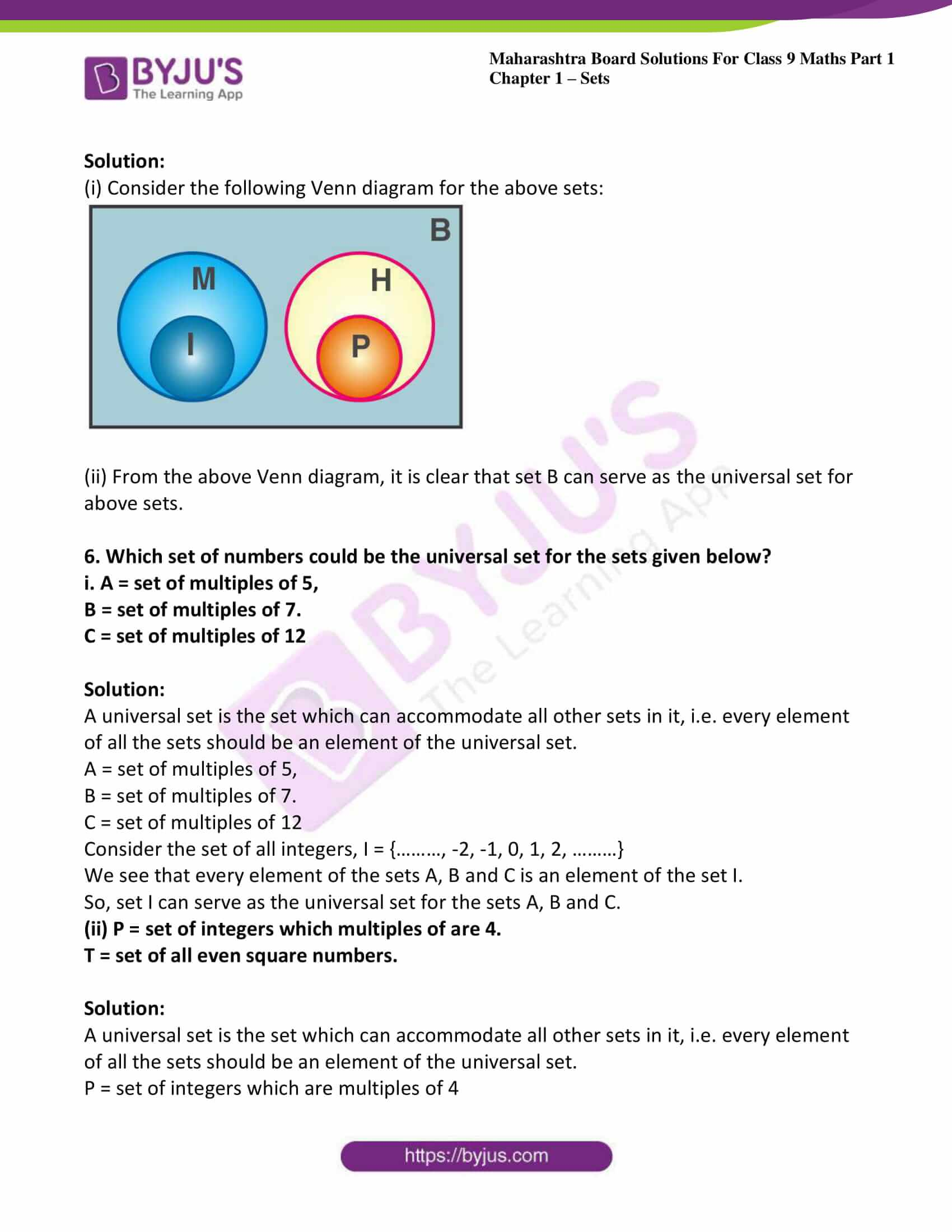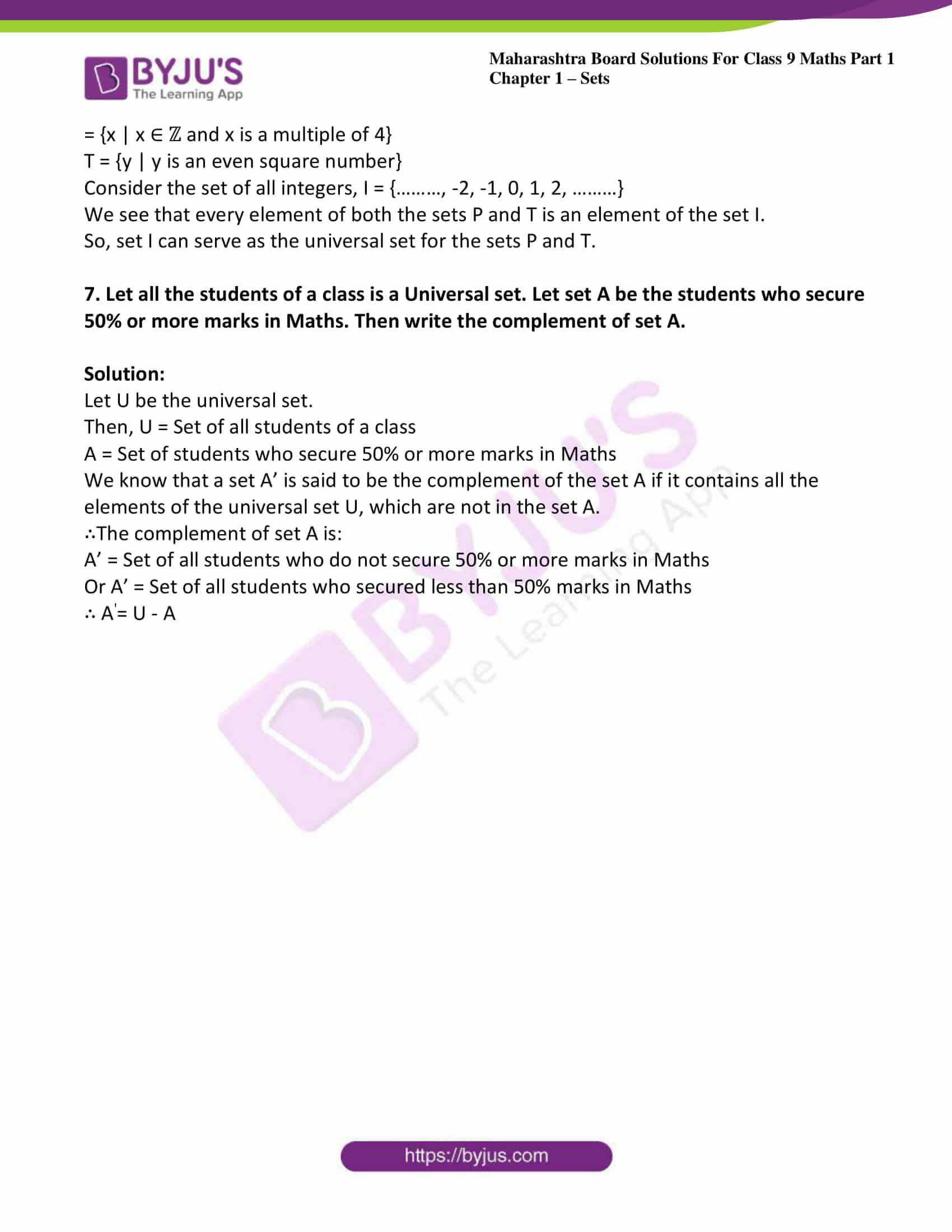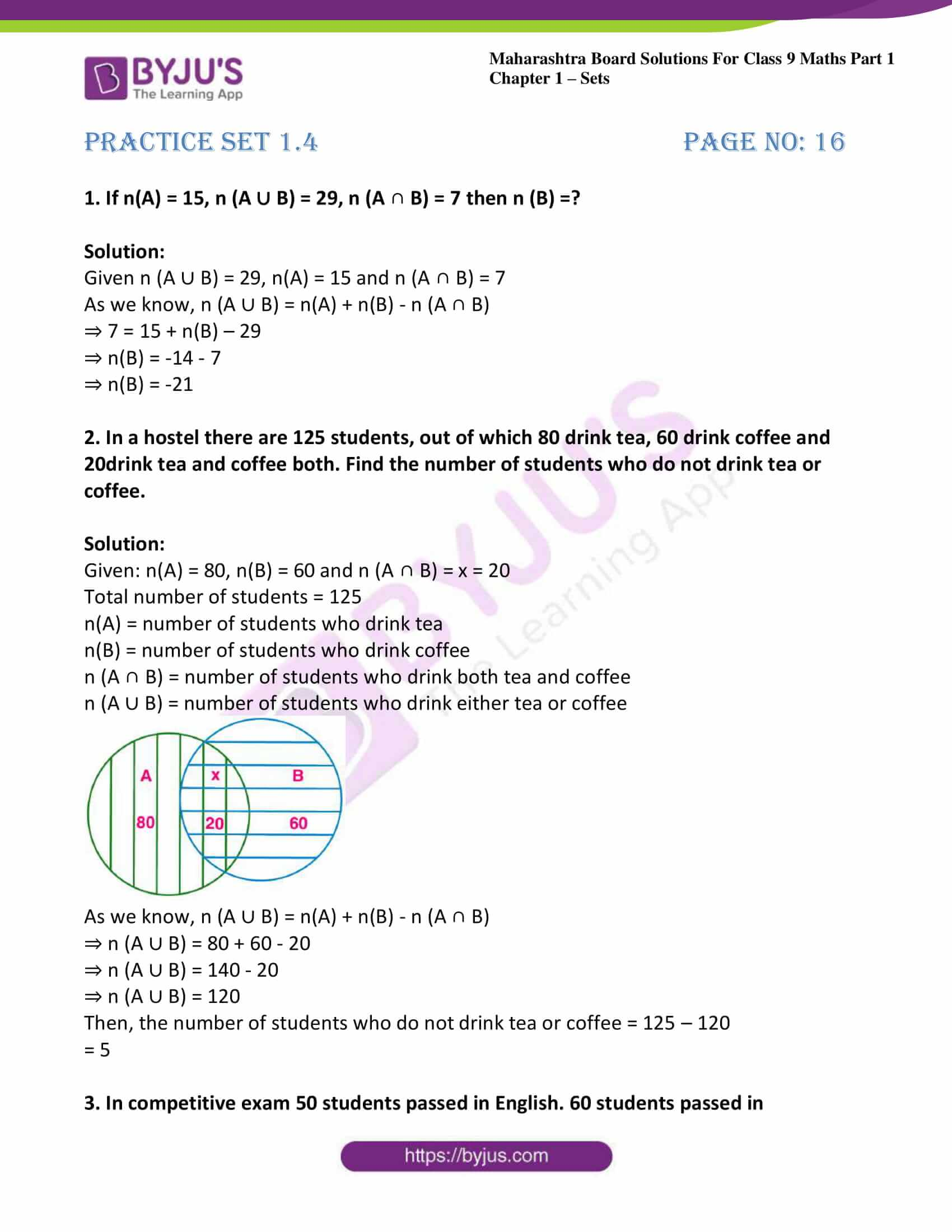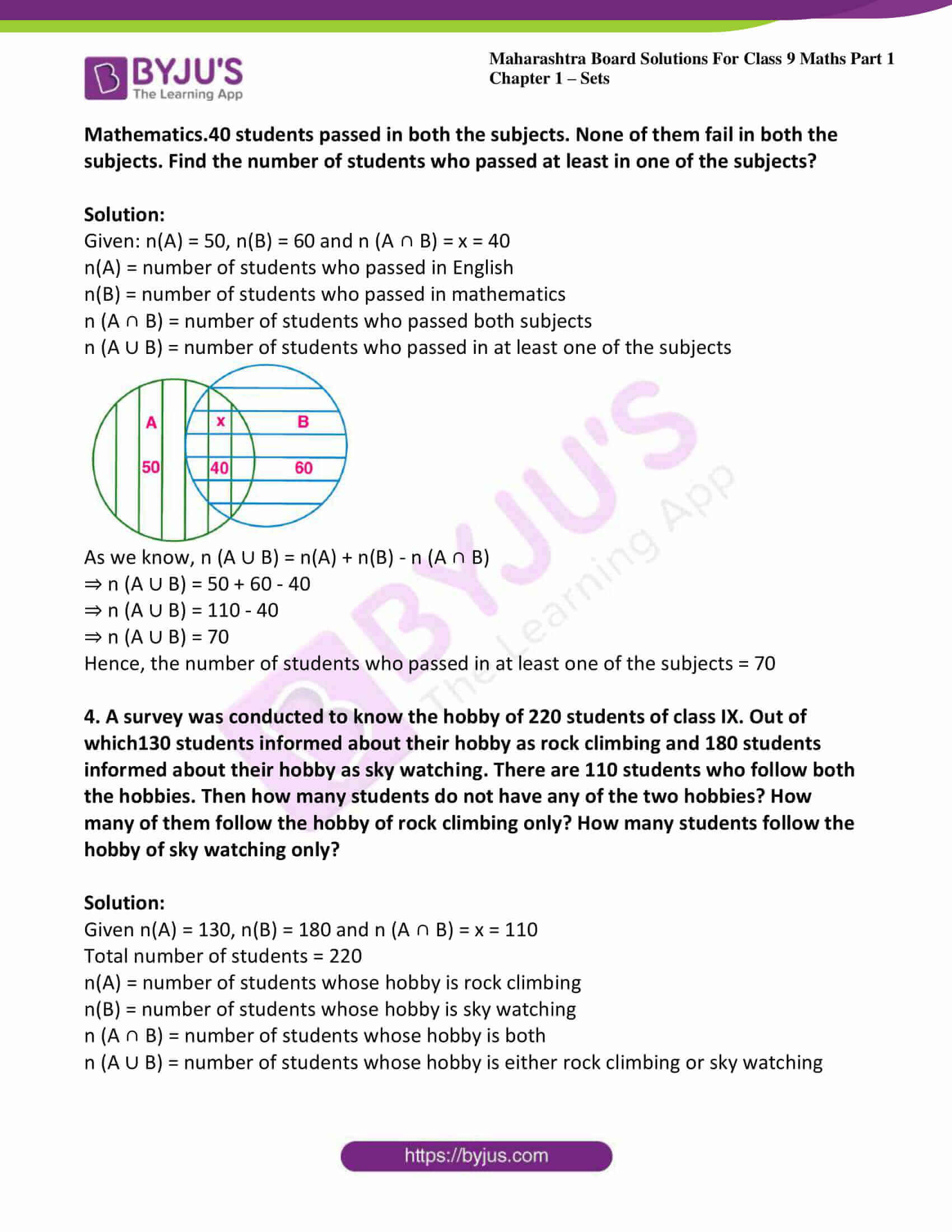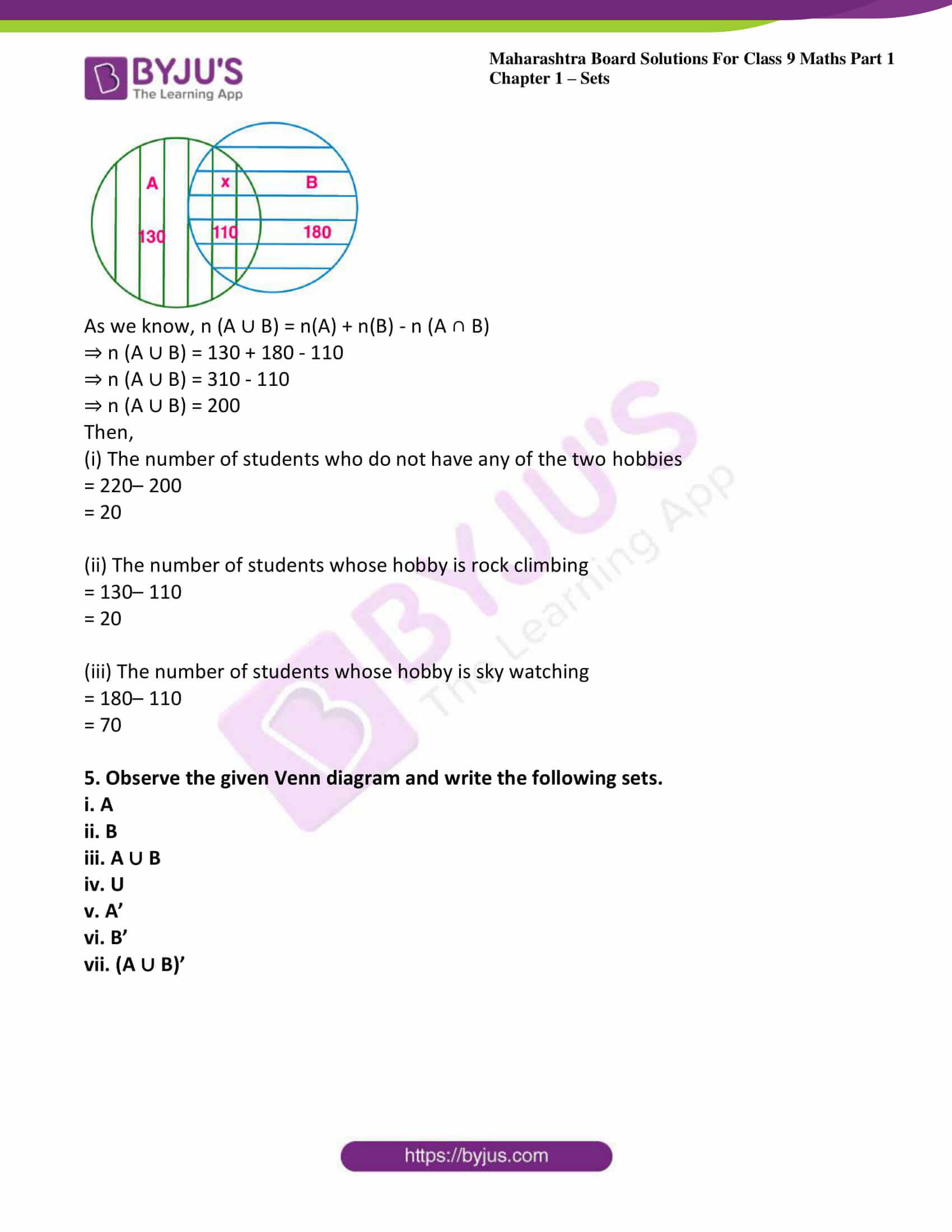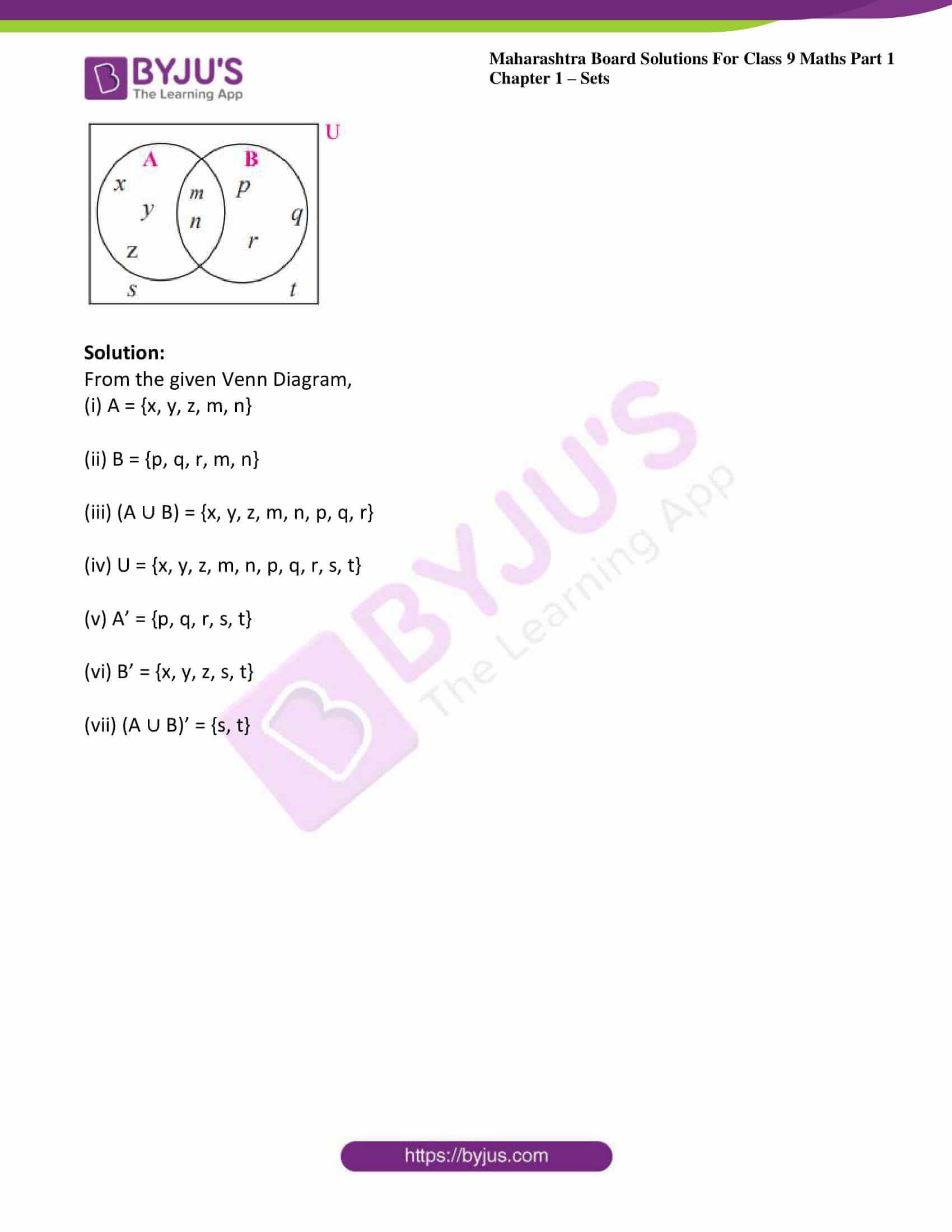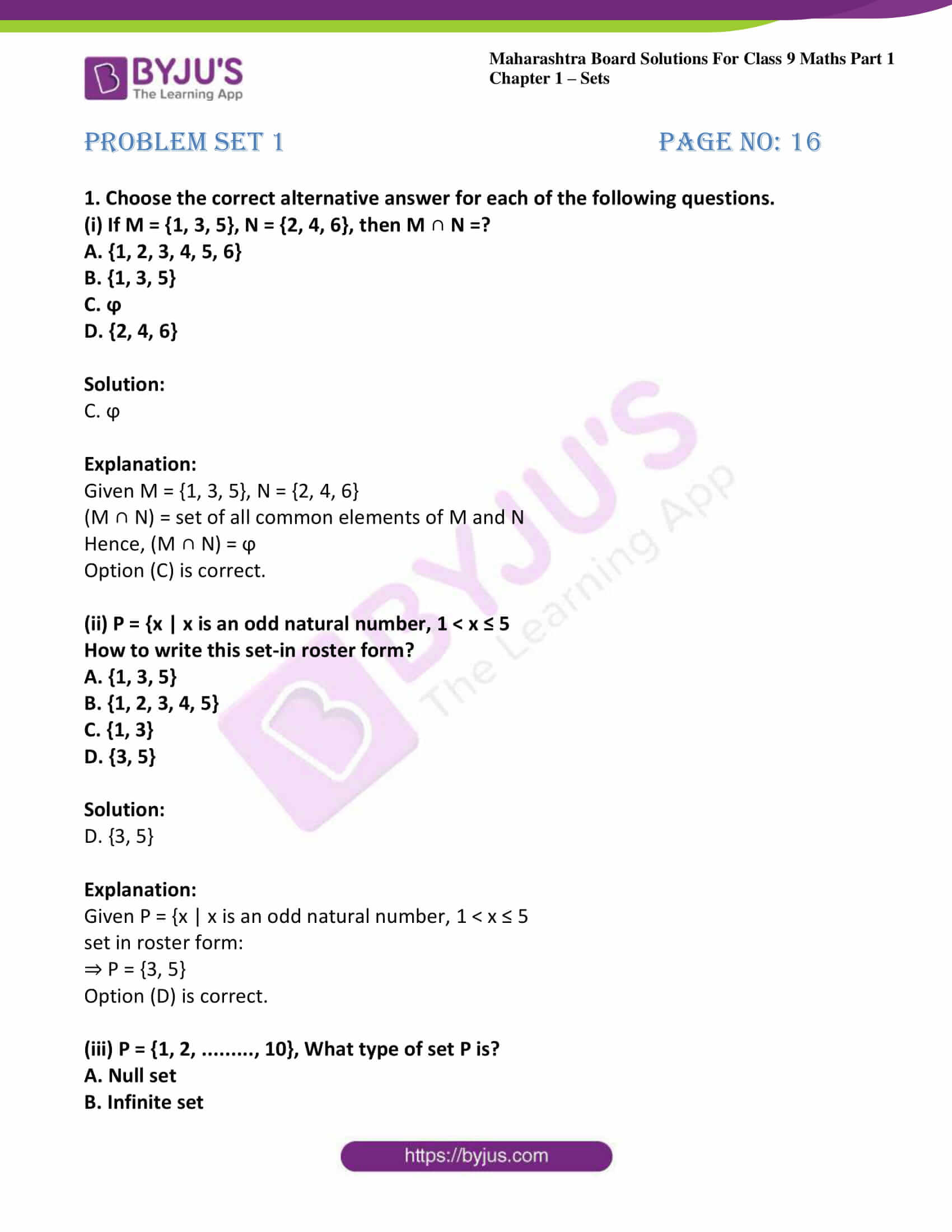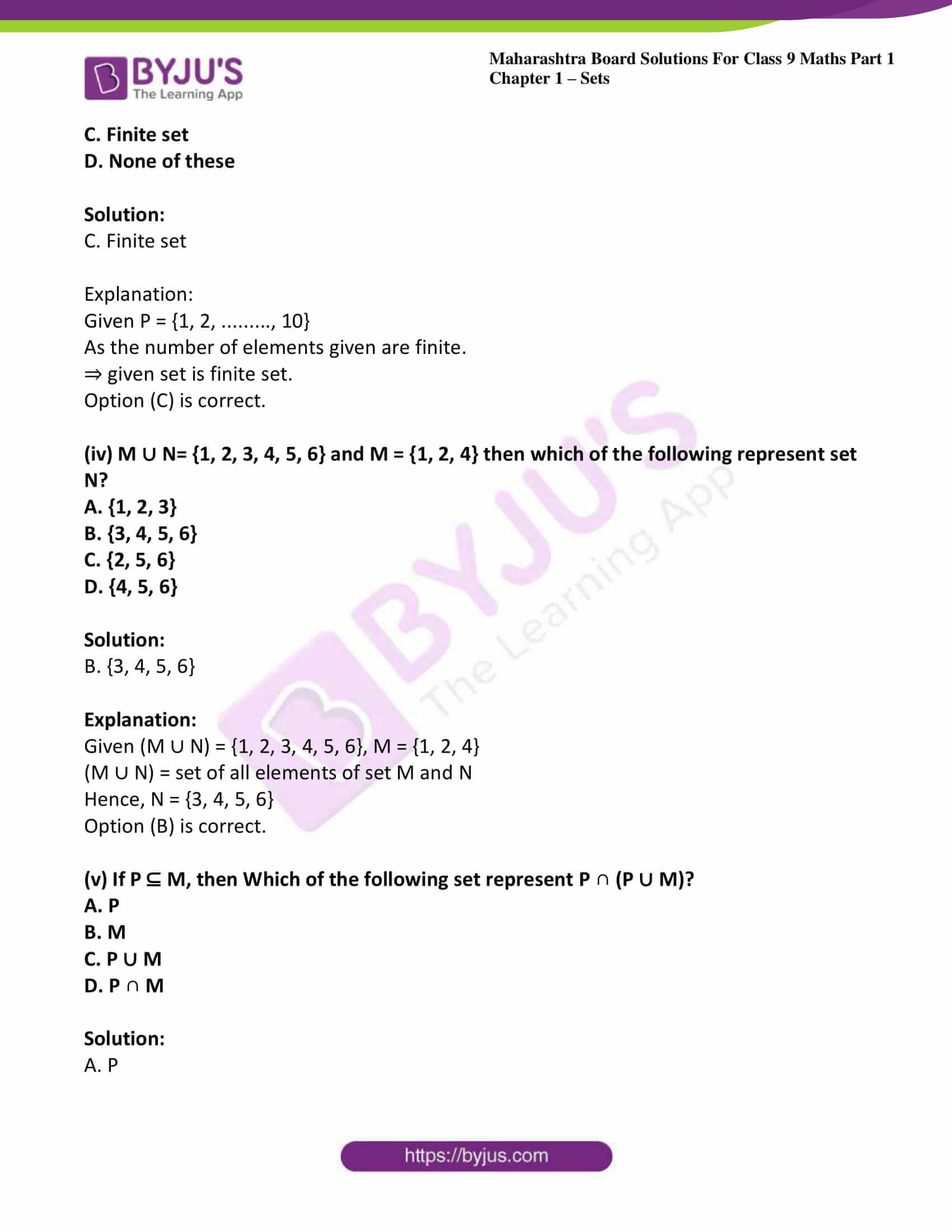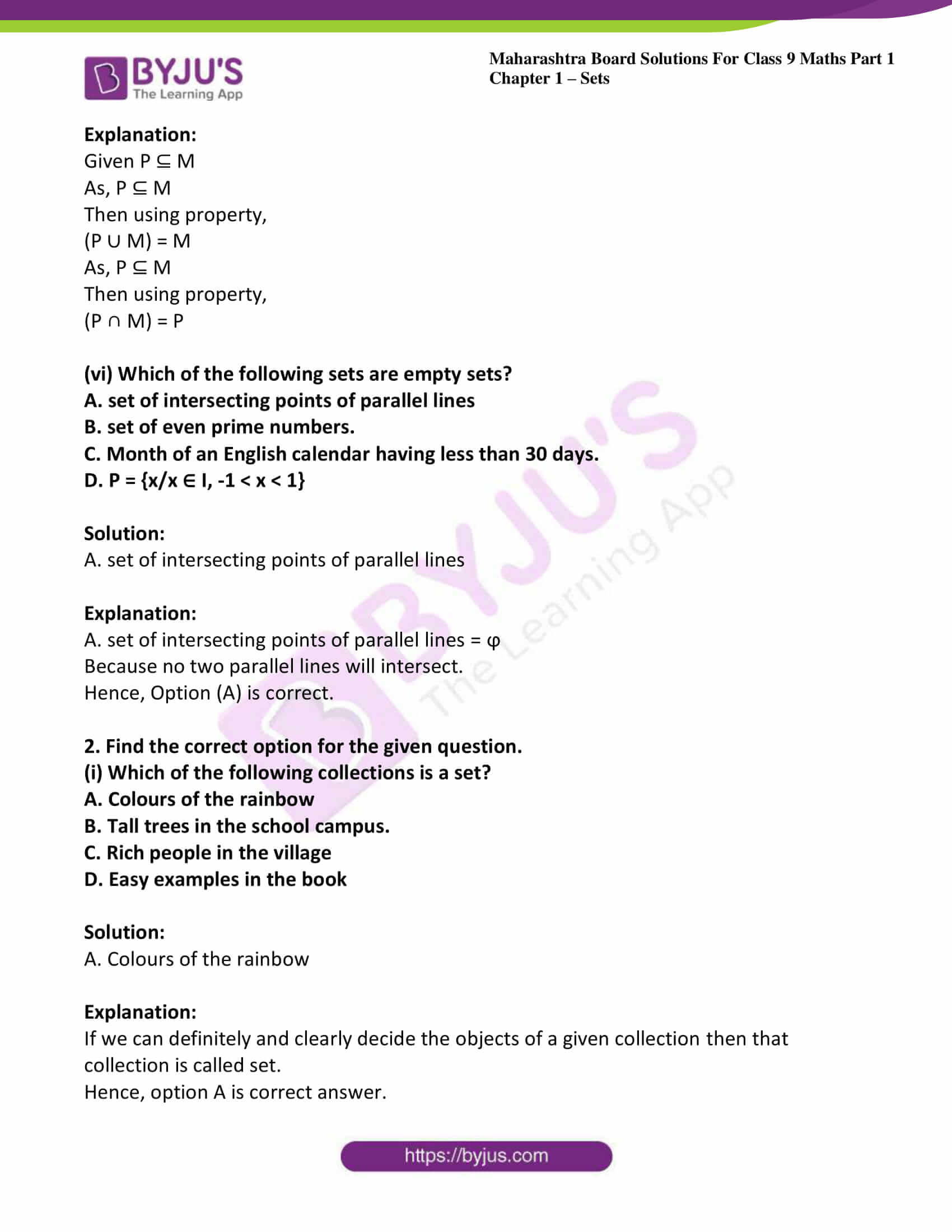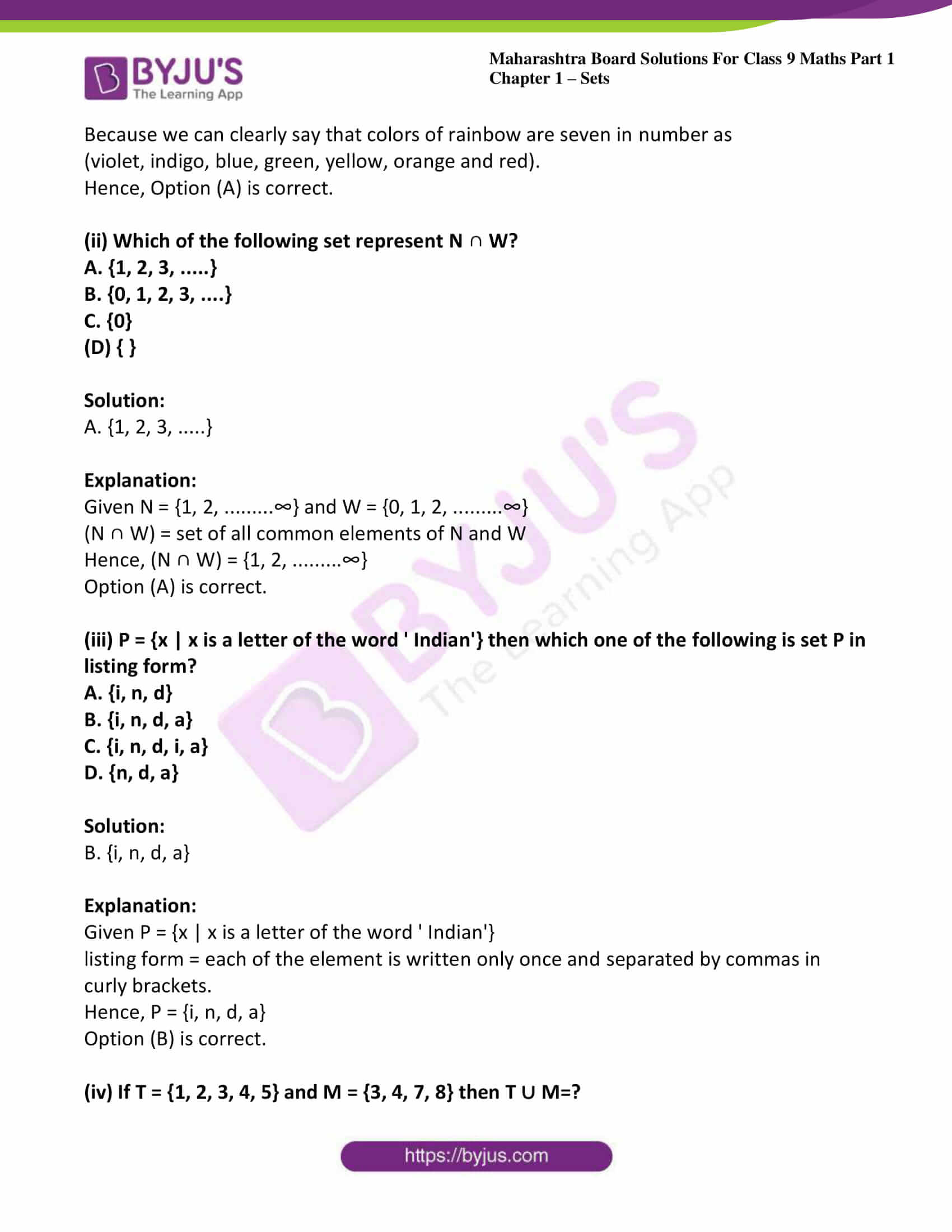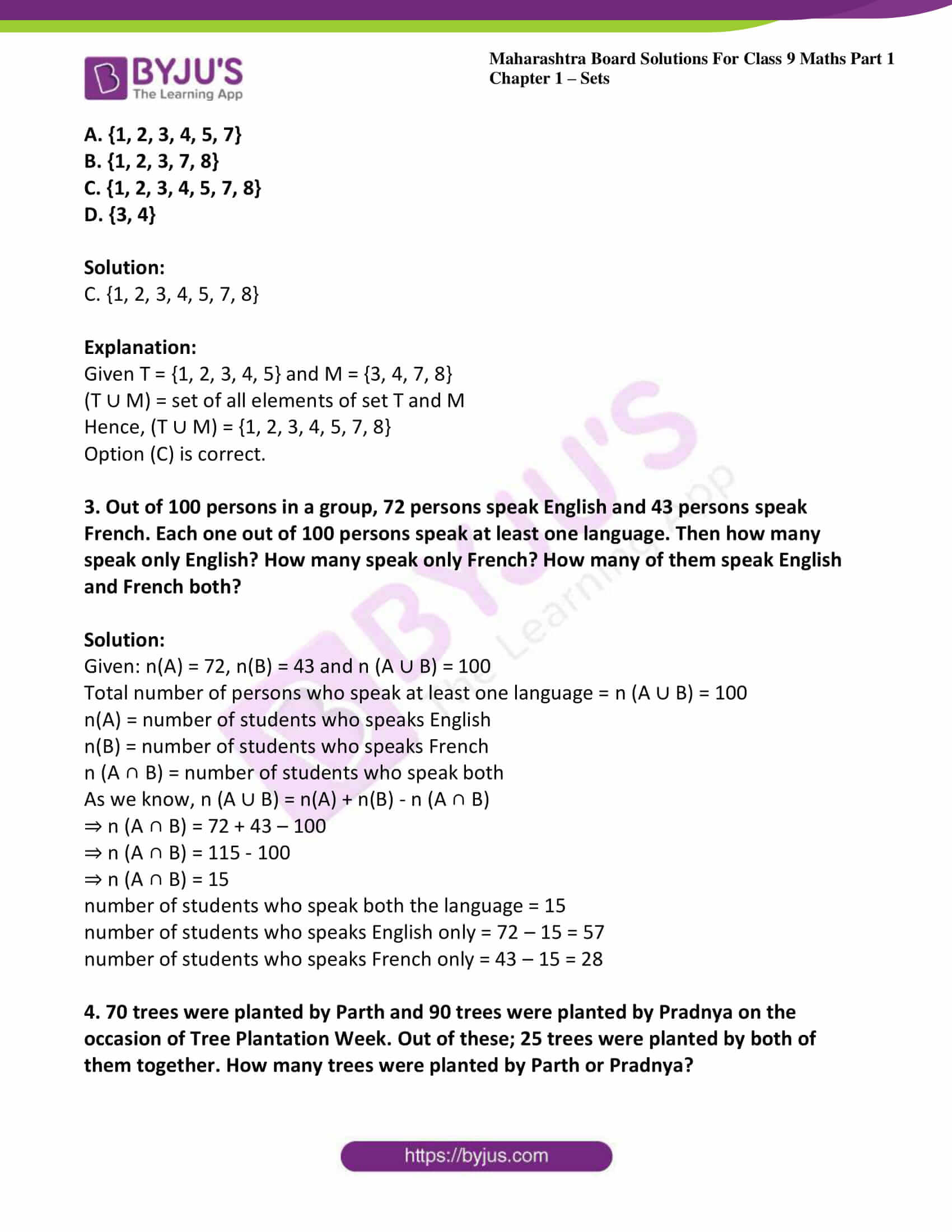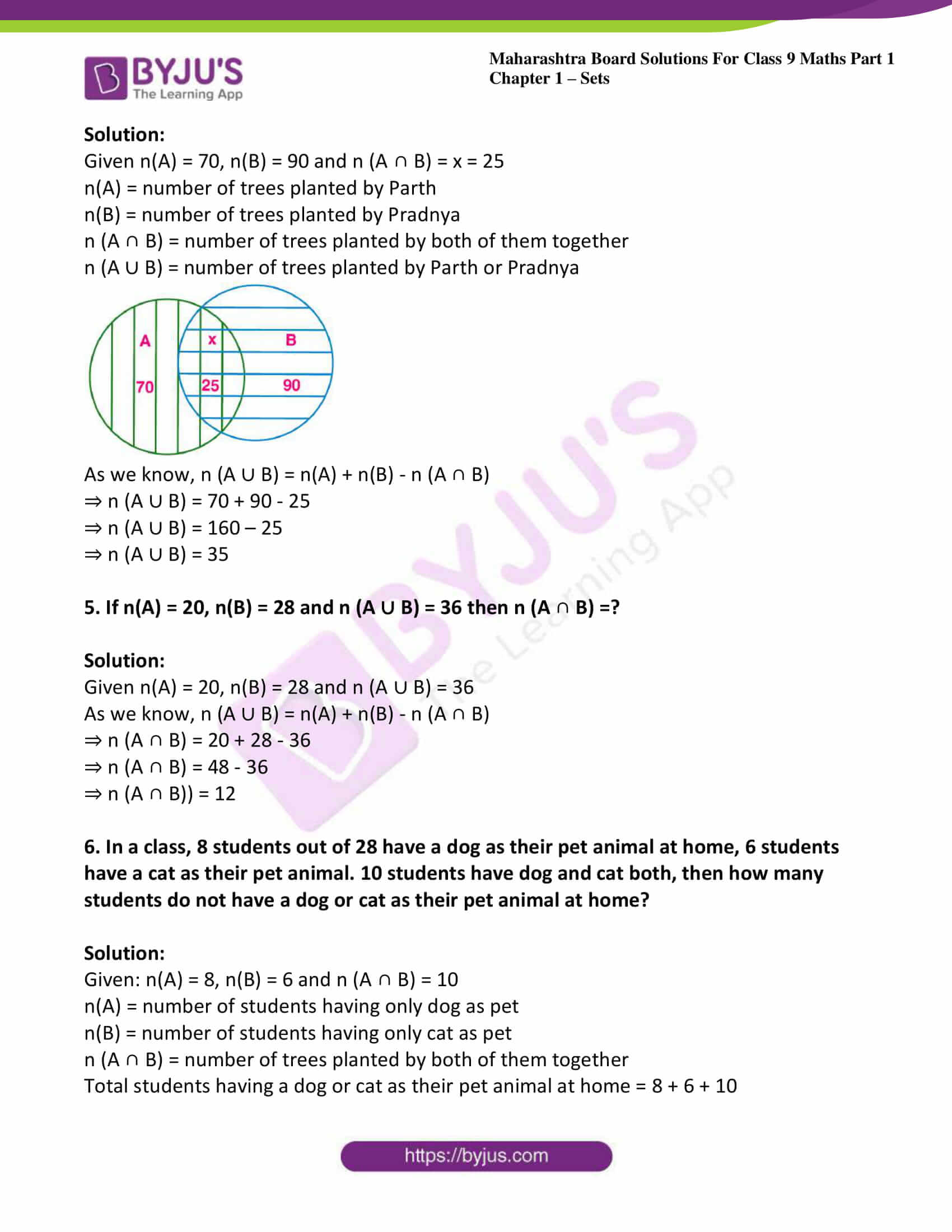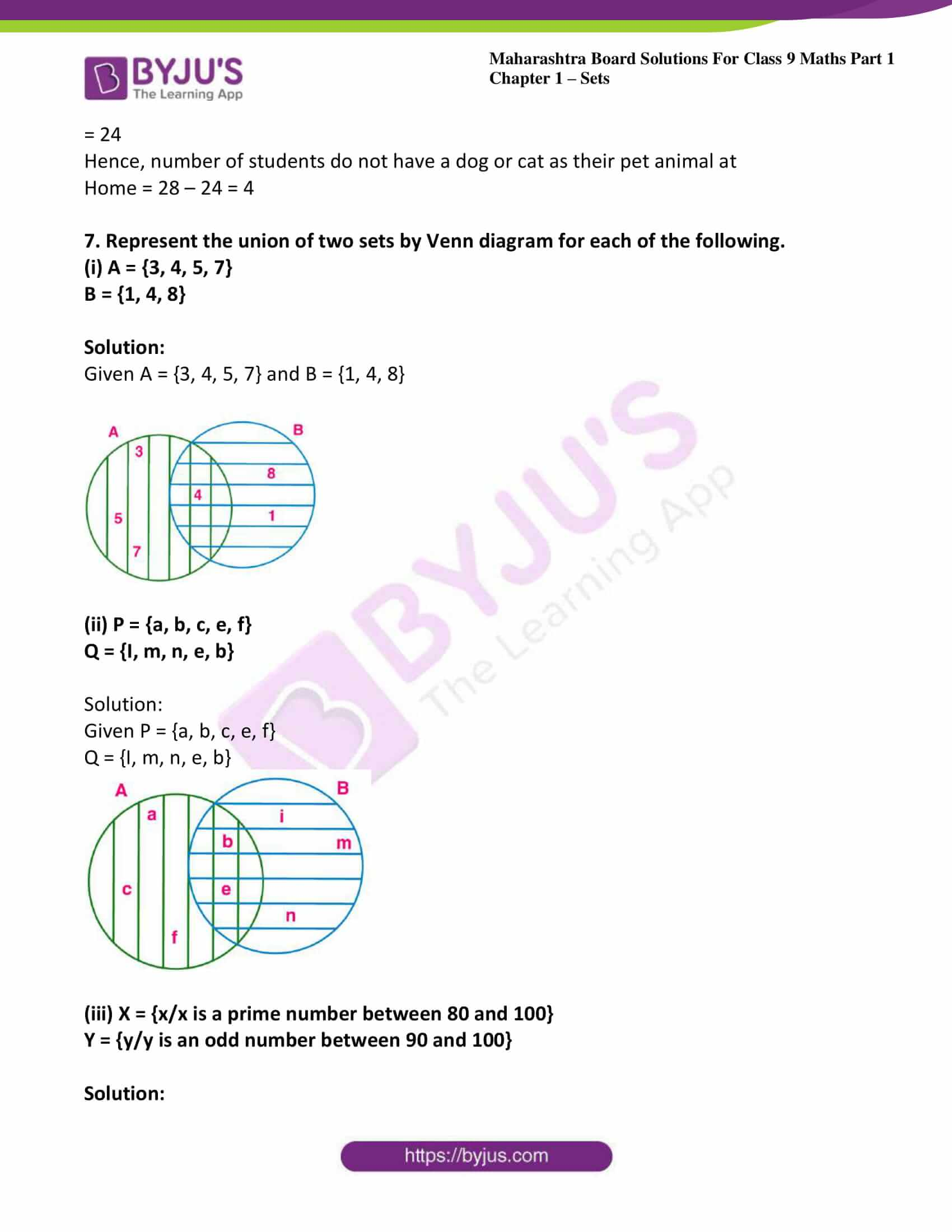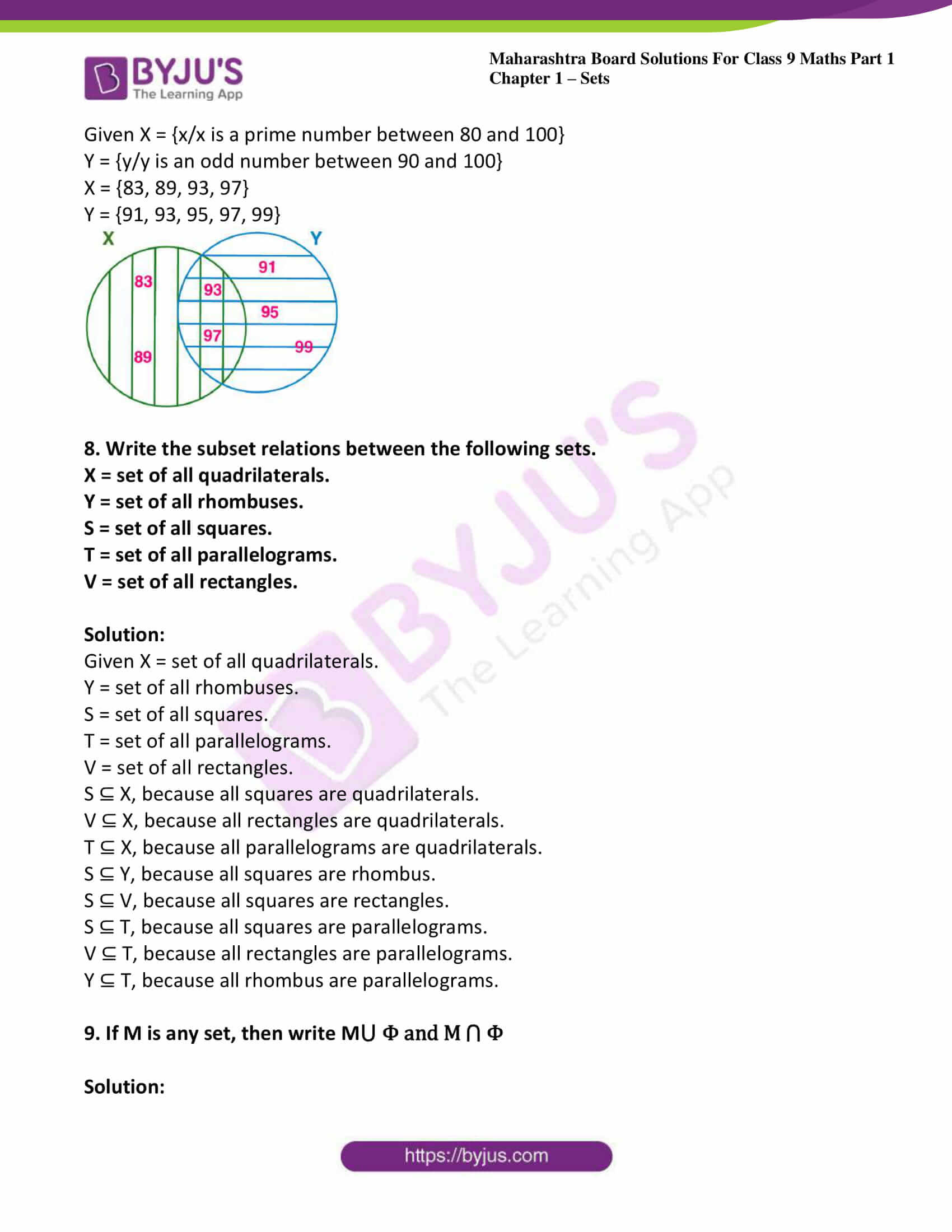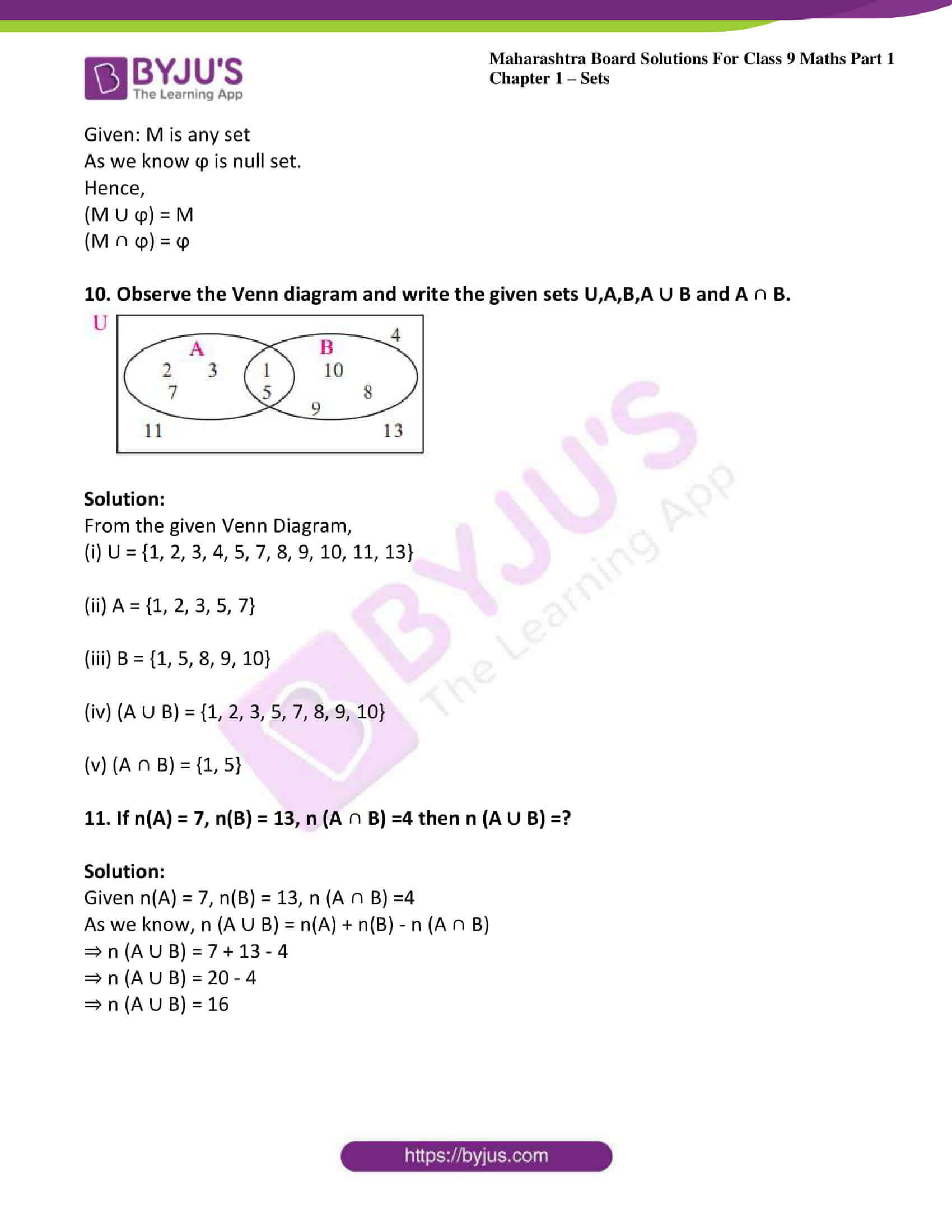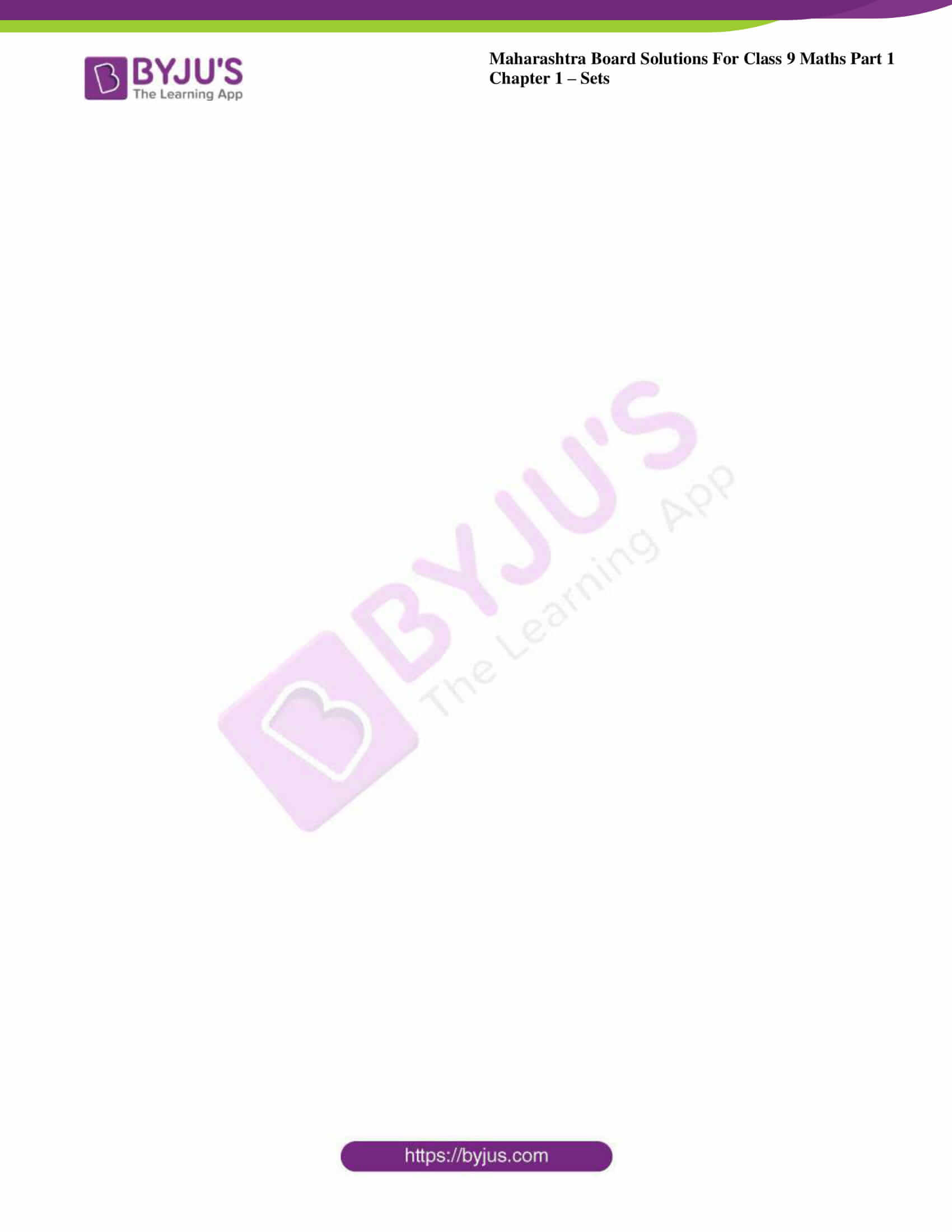### Access answers to Maths MSBSHSE Solutions For Class 9 Part 1 Chapter 1 – Sets

Practice set 1.1 Page no: 3

1. Write the following sets in roster form.
i. Set of even numbers

Solution:

We know that in roster method, we write all the elements of a set in curly bracket. Each of the element is written only once and separated by commas.

P = {2, 4, 6, 8, 10, 12, 14, …}

ii. Set of even prime numbers from 1 to 50

Solution:

We know that in roster method, we write all the elements of a set in curly bracket. Each of the element is written only once and separated by commas.

Q = {2}

iii. Set of negative integers

Solution:

We know that in roster method, we write all the elements of a set in curly bracket. Each of the element is written only once and separated by commas.

R = {… -7, -6, -5, -4, -3, -2, -1}

iv. Seven basic sounds of a sargam (sur)

Solution:

We know that in roster method, we write all the elements of a set in curly bracket. Each of the element is written only once and separated by commas.

S = {Sa, Re, Ga, Ma, Pa, Dha, Ni}

2. Write the following symbolic statements in words.

i. 4/3 ∈ Q

Solution:

We know that Q is denoted as set of rational numbers.

We write 4/3 ∈ Q as 4/3 is an element of set of rational numbers.

ii. – 2 N

Solution:

We know that N is denoted as set of natural numbers.

We write -2 N as ‘-2 is not an element of set of natural numbers.’

iii. P = {P is an odd number}

Solution:

We write P = {p| p is an odd number} as Set P contains all the odd numbers.

3. Write any two sets by listing method and by rule method.

Solution:

We know that in listing method, we write all the elements of a set in curly bracket. Each of the elements is written only once and separated by commas.

A = {2, 4, 6, 8, 10}

B = {a, e, i, o, u}

In rule method, we do not write the list of elements but write the general element using variable followed by a vertical line or colon and write the property of the variable.

A = {x/x is a multiple of 2}

B = {y/y is a vowel}

4. Write the following sets using listing method.

i. All months in the Indian solar year.

Solution:

We know that in listing method, we write all the elements of a set in curly bracket. Each of the elements is written only once and separated by commas.

A = {Chaitra, Vaishakha, Jyeshth, Ashadha, Shravan, Bhadra, Ashwin, Kartika, Agrahayan, Paush, Magh, Phalgun}

ii. Letters in the word ‘COMPLEMENT’.

Solution:

We know that in listing method, we write all the elements of a set in curly bracket. Each of the elements is written only once and separated by commas.

B = {C, O, M, P, L, E, N, T}

iii. Set of human sensory organs

Solution:

We know that in listing method, we write all the elements of a set in curly bracket. Each of the elements is written only once and separated by commas.

C = {Eyes, Ears, Nose, Mouth, Skin}

iv. Set of prime numbers from 1 to 20.

Solution:

We know that in listing method, we write all the elements of a set in curly bracket. Each of the elements is written only once and separated by commas.

D = {2, 3, 5, 7, 11, 13, 17, 19}

v. Names of continents of the world.

Solution:

We know that in listing method, we write all the elements of a set in curly bracket. Each of the elements is written only once and separated by commas.

E = {Asia, Africa, North America, South America, Antarctica, Europe, Australia}

5. Write the following sets using rule method.
i. A = {1,4,9,16,25,36,49,64,81,100}

Solution:

We know that in rule method, we do not write the list of elements but write the general element using variable followed by a vertical line or colon and write the property of the variable.

A = {x| x is a perfect square number from 1 to 101}

ii. B = {6,12,18,24,30,36,42,48}

Solution:

We know that in rule method, we do not write the list of elements but write the general element using variable followed by a vertical line or colon and write the property of the variable.

B = {y| y is a multiple of 6 and is less than 50}

iii. C = {S, M, I, L, E}

Solution:

We know that in rule method, we do not write the list of elements but write the general element using variable followed by a vertical line or colon and write the property of the variable.

C = {z| z is a letter of the world ‘SMILE’.}

iv. D = {Sunday, Monday, Tuesday, Wednesday, Thursday, Friday, Saturday}

Solution:

We know that in rule method, we do not write the list of elements but write the general element using variable followed by a vertical line or colon and write the property of the variable.

D = {p | p is a day of the week}

Practice set 1.2 Page no: 6

1. Decide which of the following are equal sets and which are not? Justify your answer.
A = {x|3x – 1 = 2}
B = {x | x is a natural number but x is neither prime nor composite}
C = {x |x ∈ N, x < 2}

Solution:

We know that two sets A and B are said to be equal, if every element of set A is in set B and every element of set B is in set A. It is symbolically written as A = B.

A = {x|3x – 1 = 2}

⇒ 3x – 1 = 2

⇒ 3x = 2 + 1 = 3

⇒ x = 3/3 = 1

∴ A = {1}

B = {x |x is a natural number but x is neither prime nor composite}

We know that 1 is neither prime nor composite and is a natural number.

∴ B = {1}

C = {x |x ∈ N, x < 2}

We know that natural number, N = {0, 1, 2, 3, …}

∴ C = {0, 1}

A = B, B ≠ C, A ≠ C

Therefore, A and B are equal sets but B and C (and) A and C are not equal sets.

2. Decide whether set A and B are equal sets. Give reason for your answer.
A = Even prime numbers
B = {x| 7x – 1 = 13}

Solution:

We know that two sets A and B are said to be equal, if every element of set A is in set B and every element of set B is in set A. It is symbolically written as A = B.

A = Even Prime Numbers = {2}

B = {x| 7x – 1 = 13}

⇒ 7x – 1 = 13

⇒ 7x = 13 + 1 = 14

⇒ x = 14/7

∴ x = 2

∴ A = B

Therefore, Set A and set B are equal sets.

3. Which of the following are empty sets? Why?
i. A = {a |a is a natural number smaller than zero.}

Solution:

We know that If there is not a single element in the set which satisfies the given condition then it is called a Null set or an Empty set. Empty set is represented by { }.

We know that Natural number, N = {0, 1, 2, … }.

∴ A = {a |a is a natural number smaller than zero} = {}

Therefore, A is an empty set.

ii. B = {x|x2 = 0}

Solution:

Given

B = {x|x2 = 0}

⇒ x2 = 0

∴ x = 0

B = {x|x2 = 0} = {0} ≠ {}

Therefore, B is not an empty set.

iii. C = {x|5x – 2 = 0, x ∈ N}

Solution:

Given C = {x|5x – 2 = 0, x ∈ N}

⇒ 5x – 2 = 0

⇒ 5x = 2

⇒ x = 5/2

We know that natural number, N = {0, 1, 2, …}

∴ x N

∴ C = {x|5x – 2 = 0, x ∈ N} = {}

Therefore, C is an empty set.

4. Write with reasons, which of the following sets are finite or infinite. We know that if a set is a null set or number of elements are limited and countable then it is called ‘Finite set’ and if number of elements in a set is unlimited and uncountable then the set is called ‘Infinite set’.
i. A = {x|x < 10, x is a natural number}
ii. B = {y |y< -1, is an integer}
iii. C = Set of students of class 9 from your school.
iv. Set of people from your village.
v. Set of apparatus in laboratory
vi. Set of whole numbers
vii. Set of rational number

Solution:

i. A = {x|x < 10, x is a natural number}

∴ A = {0, 1, 2, 3, 4, 5, 6, 7, 8, 9}

Therefore, A is a finite set.

ii. B = {y |y< -1, y is an integer}

∴ B = {… -5, -4, -3, -2}

Therefore, B is an infinite set.

iii. C = {a, b, c, d, e, …}

Therefore, C is an infinite set.

iv. D = {Sarpanch, villager1, villager2, …}

Therefore, D is an infinite set.

v. E = {Beakers, funnels, chemicals, thermometers, …}

Therefore, E is an infinite set.

vi. W = {1, 2, 3, 4, 5, …}

Therefore, W is an infinite set.

vii. Q = {(p/q) |p, q ∈ I, q ≠ 0}

Q = {1/2, 1/3, 1/4, …}

Therefore, Q is an infinite set.

Practice set 1.3 Page no: 11

1. If A = {a, b, c, d, e}, B = {c, d, e, f}, C = {b, d} and D = {a, e}.
then which of the following statements are true and which are false?
i. C ⊆ B

ii. A ⊆ D
iii. D ⊆ B

iv. D ⊆ A
v. B ⊆ A

vi. C ⊆ A

Solution:

Consider the following Venn diagram to check whether the above statements are true or not.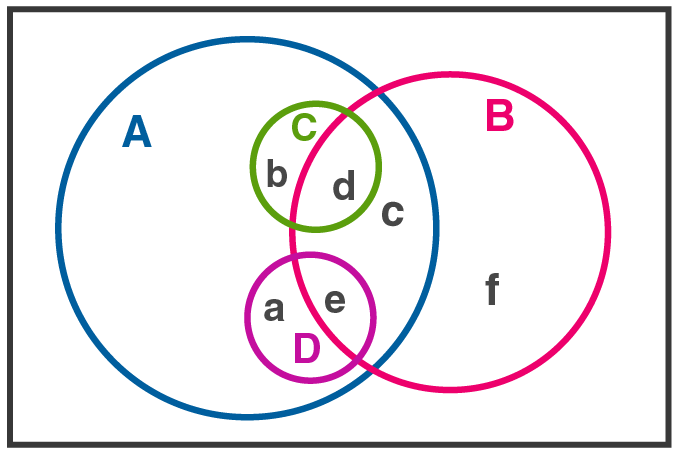Given A = {a, b, c, d, e}, B = {c, d, e, f}, C = {b, d} and D = {a, e}.

So, it is clear from above that every element of set C and set D is also an element of set A.

∴ C ⊆ A and D ⊆ A

Hence, (iv), (vi) are true and (ii) is false.

Also, it is clear from above that every element of set B is not an element of set A.

∴ (v) is false.

Similarly, it is clear that every element of set C is not an element of set B.

∴ (i) is false.

Also, every element of set D is not an element of set B.

∴ (iii) is false.

2. Take the set of natural numbers from 1 to 20 as universal set and show set X and Y using Venn diagram.
i. X = {x | x ∈ ℕ, and 7 < x < 15}
ii. Y = {y | y ∈ ℕ, y is prime number from 1 to 20}

Solution: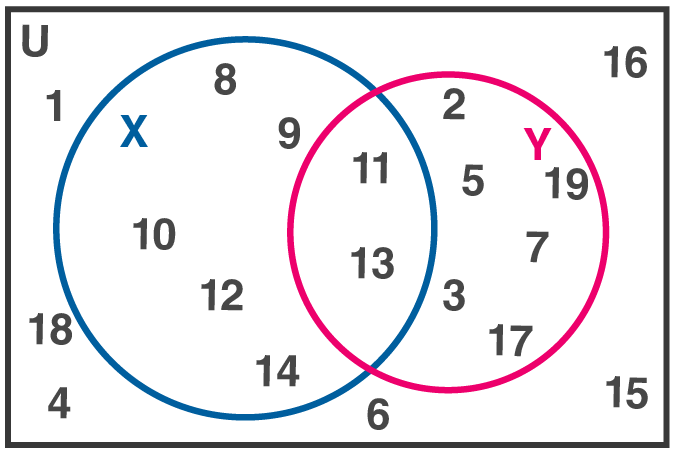From the Venn diagram we can see that,

Consider the universal set:

U = {u | u ∈ ℕ, 1 ≤ x ≤ 20}

= {1, 2, 3, 4, 5, 6, 7, 8, 9, 10, 11, 12, 13, 14, 15, 16, 17, 18, 19, 20}

Given that

X = {x | x ∈ ℕ, 7 < x < 15}

= {8, 9, 10, 11, 12, 13, 14}

Also given that

Y = {y | y ∈ ℕ, y is prime number from 1 to 20}

= {2, 3, 5, 7, 11, 13, 17, 19}

3. U = {1, 2, 3, 7, 8, 9, 10, 11, 12}
P = {1, 3, 7, 10}
then (i) show the sets U, P and P’ by Venn diagram. (ii) Verify (P’)’ = P

Solution:

U = {1, 2, 3, 7, 8, 9, 10, 11, 12}

P = {1, 3, 7, 10}

P’ = {x | x ∈ U and x ∉ P} = {2, 8, 9, 11, 12}

(i) The Venn diagram showing U, P and P’ is: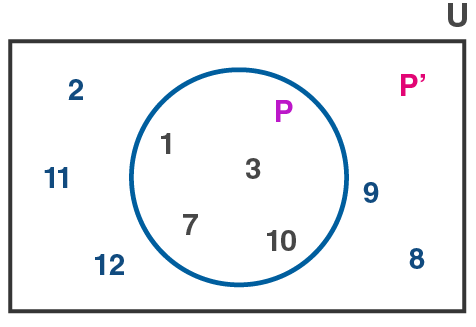(ii) Now, we consider (P’)’

(P’)’ = {x | x ∈ U and x ∉ P’}

= {1, 3, 7, 10}

= P

Hence, (P’)’ = P.

4. A = {1, 3, 2, 7} then write any three subsets of A.

Solution:

We know that any set S such that every element of S is an element of the set A is said to be a subset of A.

∴ Consider B = {1, 2, 3}, C = {1, 3} and D = {2, 3, 7}

It is clear from above that every element of the sets B, C and D is an element of set A, so, B, C and D are subsets of A.

Also, note that

Every set is a subset of itself, i. e. A ⊆ A and

Empty set is a subset of every set, i. e. ϕ ⊆ A.

So, A and ϕ are also subsets of A.

5. (i) Write the subset relation between the sets.
P is the set of all residents in Pune.
I is the set of all residents in Indore.
B is the set of all residents in India.
H is the set of all residents in Maharashtra.
(ii) Which set can be the universal set for above sets?

Solution:

(i) Consider the following Venn diagram for the above sets: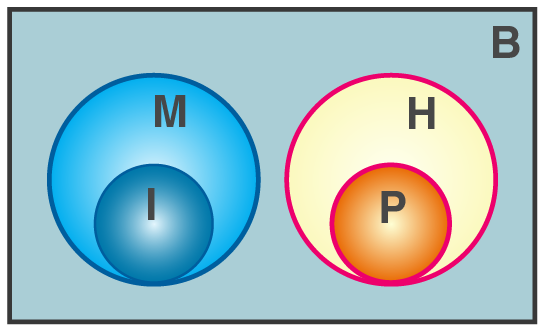(ii) From the above Venn diagram, it is clear that set B can serve as the universal set for above sets.

6. Which set of numbers could be the universal set for the sets given below?
i. A = set of multiples of 5,
B = set of multiples of 7.
C = set of multiples of 12

Solution:

A universal set is the set which can accommodate all other sets in it, i.e. every element of all the sets should be an element of the universal set.

A = set of multiples of 5,

B = set of multiples of 7.

C = set of multiples of 12

Consider the set of all integers, I = {………, -2, -1, 0, 1, 2, ………}

We see that every element of the sets A, B and C is an element of the set I.

So, set I can serve as the universal set for the sets A, B and C.

(ii) P = set of integers which multiples of are 4.
T = set of all even square numbers.

Solution:

A universal set is the set which can accommodate all other sets in it, i.e. every element of all the sets should be an element of the universal set.

P = set of integers which are multiples of 4

= {x | x ∈ ℤ and x is a multiple of 4}

T = {y | y is an even square number}

Consider the set of all integers, I = {………, -2, -1, 0, 1, 2, ………}

We see that every element of both the sets P and T is an element of the set I.

So, set I can serve as the universal set for the sets P and T.

7. Let all the students of a class is a Universal set. Let set A be the students who secure 50% or more marks in Maths. Then write the complement of set A.

Solution:

Let U be the universal set.

Then, U = Set of all students of a class

A = Set of students who secure 50% or more marks in Maths

We know that a set A’ is said to be the complement of the set A if it contains all the elements of the universal set U, which are not in the set A.

∴The complement of set A is:

A’ = Set of all students who do not secure 50% or more marks in Maths

Or A’ = Set of all students who secured less than 50% marks in Maths

∴ A= U – A

Practice set 1.4 Page no: 16

1. If n(A) = 15, n (A ∪ B) = 29, n (A ∩ B) = 7 then n (B) =?

Solution:

Given n (A ∪ B) = 29, n(A) = 15 and n (A ∩ B) = 7

As we know, n (A ∪ B) = n(A) + n(B) – n (A ∩ B)

⇒ 7 = 15 + n(B) – 29

⇒ n(B) = -14 – 7

⇒ n(B) = -21

2. In a hostel there are 125 students, out of which 80 drink tea, 60 drink coffee and 20drink tea and coffee both. Find the number of students who do not drink tea or coffee.

Solution:

Given: n(A) = 80, n(B) = 60 and n (A ∩ B) = x = 20

Total number of students = 125

n(A) = number of students who drink tea

n(B) = number of students who drink coffee

n (A ∩ B) = number of students who drink both tea and coffee

n (A ∪ B) = number of students who drink either tea or coffee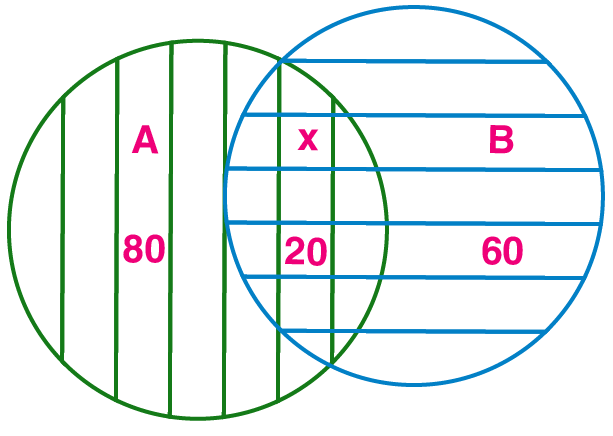As we know, n (A ∪ B) = n(A) + n(B) – n (A ∩ B)

⇒ n (A ∪ B) = 80 + 60 – 20

⇒ n (A ∪ B) = 140 – 20

⇒ n (A ∪ B) = 120

Then, the number of students who do not drink tea or coffee = 125 – 120

= 5

3. In competitive exam 50 students passed in English. 60 students passed in Mathematics.40 students passed in both the subjects. None of them fail in both the subjects. Find the number of students who passed at least in one of the subjects?

Solution:

Given: n(A) = 50, n(B) = 60 and n (A ∩ B) = x = 40

n(A) = number of students who passed in English

n(B) = number of students who passed in mathematics

n (A ∩ B) = number of students who passed both subjects

n (A ∪ B) = number of students who passed in at least one of the subjects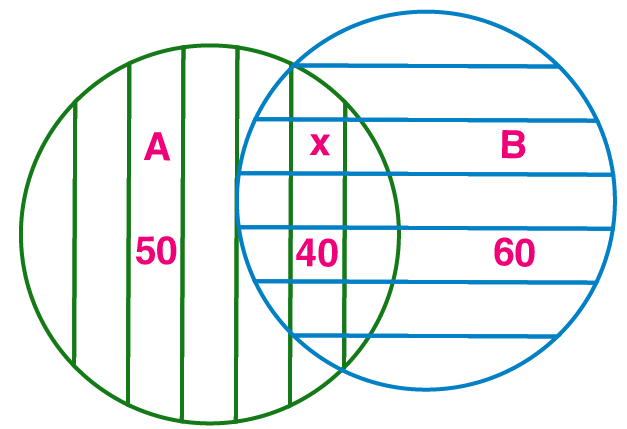As we know, n (A ∪ B) = n(A) + n(B) – n (A ∩ B)

⇒ n (A ∪ B) = 50 + 60 – 40

⇒ n (A ∪ B) = 110 – 40

⇒ n (A ∪ B) = 70

Hence, the number of students who passed in at least one of the subjects = 70

4. A survey was conducted to know the hobby of 220 students of class IX. Out of which130 students informed about their hobby as rock climbing and 180 students informed about their hobby as sky watching. There are 110 students who follow both the hobbies. Then how many students do not have any of the two hobbies? How many of them follow the hobby of rock climbing only? How many students follow the hobby of sky watching only?

Solution:

Given n(A) = 130, n(B) = 180 and n (A ∩ B) = x = 110

Total number of students = 220

n(A) = number of students whose hobby is rock climbing

n(B) = number of students whose hobby is sky watching

n (A ∩ B) = number of students whose hobby is both

n (A ∪ B) = number of students whose hobby is either rock climbing or sky watching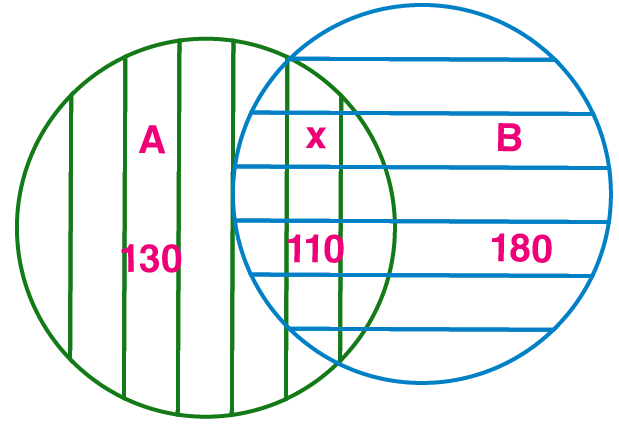As we know, n (A ∪ B) = n(A) + n(B) – n (A ∩ B)

⇒ n (A ∪ B) = 130 + 180 – 110

⇒ n (A ∪ B) = 310 – 110

⇒ n (A ∪ B) = 200

Then,

(i) The number of students who do not have any of the two hobbies

= 220– 200

= 20

(ii) The number of students whose hobby is rock climbing

= 130– 110

= 20

(iii) The number of students whose hobby is sky watching

= 180– 110

= 70

5. Observe the given Venn diagram and write the following sets.
i. A

ii. B
iii. A ∪ B

iv. U
v. A’

vi. B’
vii. (A ∪ B)’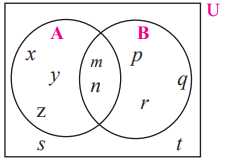Solution:

From the given Venn Diagram,

(i) A = {x, y, z, m, n}

(ii) B = {p, q, r, m, n}

(iii) (A ∪ B) = {x, y, z, m, n, p, q, r}

(iv) U = {x, y, z, m, n, p, q, r, s, t}

(v) A’ = {p, q, r, s, t}

(vi) B’ = {x, y, z, s, t}

(vii) (A ∪ B)’ = {s, t}

Problem set 1 Page no: 16

1. Choose the correct alternative answer for each of the following questions.

(i) If M = {1, 3, 5}, N = {2, 4, 6}, then M ∩ N =?
A. {1, 2, 3, 4, 5, 6}
B. {1, 3, 5}
C. ϕ
D. {2, 4, 6}

Solution:

C. ϕ

Explanation:

Given M = {1, 3, 5}, N = {2, 4, 6}

(M ∩ N) = set of all common elements of M and N

Hence, (M ∩ N) = ϕ

Option (C) is correct.

(ii) P = {x | x is an odd natural number,
1 < x ≤ 5
How to write this set-in roster form?
A. {1, 3, 5}
B. {1, 2, 3, 4, 5}
C. {1, 3}

D. {3, 5}

Solution:

D. {3, 5}

Explanation:

Given P = {x | x is an odd natural number, 1 < x ≤ 5

set in roster form:

⇒ P = {3, 5}

Option (D) is correct.

(iii) P = {1, 2, ………, 10}, What type of set P is?
A. Null set
B. Infinite set
C. Finite set
D. None of these

Solution:

C. Finite set

Explanation:

Given P = {1, 2, ………, 10}

As the number of elements given are finite.

⇒ given set is finite set.

Option (C) is correct.

(iv) M ∪ N= {1, 2, 3, 4, 5, 6} and M = {1, 2, 4} then which of the following represent set N?
A. {1, 2, 3}
B. {3, 4, 5, 6}
C. {2, 5, 6}
D. {4, 5, 6}

Solution:

B. {3, 4, 5, 6}

Explanation:

Given (M ∪ N) = {1, 2, 3, 4, 5, 6}, M = {1, 2, 4}

(M ∪ N) = set of all elements of set M and N

Hence, N = {3, 4, 5, 6}

Option (B) is correct.

(v) If
P ⊆ M, then Which of the following set represent P ∩ (P ∪ M)?
A. P
B. M
C. P ∪ M
D. P ∩ M

Solution:

A. P

Explanation:

Given P ⊆ M

As, P ⊆ M

Then using property,

(P ∪ M) = M

As, P ⊆ M

Then using property,

(P ∩ M) = P

(vi) Which of the following sets are empty sets?
A. set of intersecting points of parallel lines
B. set of even prime numbers.
C. Month of an English calendar having less than 30 days.
D. P = {x/x ∈ I, -1 < x < 1}

Solution:

A. set of intersecting points of parallel lines

Explanation:

A. set of intersecting points of parallel lines = ϕ

Because no two parallel lines will intersect.

Hence, Option (A) is correct.

2. Find the correct option for the given question.

(i) Which of the following collections is a set?
A. Colours of the rainbow
B. Tall trees in the school campus.
C. Rich people in the village
D. Easy examples in the book

Solution:

A. Colours of the rainbow

Explanation:

If we can definitely and clearly decide the objects of a given collection then that collection is called set.

Hence, option A is correct answer.

Because we can clearly say that colors of rainbow are seven in number as

(violet, indigo, blue, green, yellow, orange and red).

Hence, Option (A) is correct.

(ii) Which of the following set represent N ∩ W?
A. {1, 2, 3, …..}
B. {0, 1, 2, 3, ….}
C. {0}
(D) { }

Solution:

A. {1, 2, 3, …..}

Explanation:

Given N = {1, 2, ………∞} and W = {0, 1, 2, ………∞}

(N ∩ W) = set of all common elements of N and W

Hence, (N ∩ W) = {1, 2, ………∞}

Option (A) is correct.

(iii) P = {x | x is a letter of the word ‘ Indian’} then which one of the following is set P in listing form?
A. {i, n, d}
B. {i, n, d, a}
C. {i, n, d, i, a}
D. {n, d, a}

Solution:

B. {i, n, d, a}

Explanation:

Given P = {x | x is a letter of the word ‘ Indian’}

listing form = each of the element is written only once and separated by commas in curly brackets.

Hence, P = {i, n, d, a}

Option (B) is correct.

(iv) If T = {1, 2, 3, 4, 5} and M = {3, 4, 7, 8} then T ∪ M=?
A. {1, 2, 3, 4, 5, 7}
B. {1, 2, 3, 7, 8}
C. {1, 2, 3, 4, 5, 7, 8}
D. {3, 4}

Solution:

C. {1, 2, 3, 4, 5, 7, 8}

Explanation:

Given T = {1, 2, 3, 4, 5} and M = {3, 4, 7, 8}

(T ∪ M) = set of all elements of set T and M

Hence, (T ∪ M) = {1, 2, 3, 4, 5, 7, 8}

Option (C) is correct.

3. Out of 100 persons in a group, 72 persons speak English and 43 persons speak French. Each one out of 100 persons speak at least one language. Then how many speak only English? How many speak only French? How many of them speak English and French both?

Solution:

Given: n(A) = 72, n(B) = 43 and n (A ∪ B) = 100

Total number of persons who speak at least one language = n (A ∪ B) = 100

n(A) = number of students who speaks English

n(B) = number of students who speaks French

n (A ∩ B) = number of students who speak both

As we know, n (A ∪ B) = n(A) + n(B) – n (A ∩ B)

⇒ n (A ∩ B) = 72 + 43 – 100

⇒ n (A ∩ B) = 115 – 100

⇒ n (A ∩ B) = 15

number of students who speak both the language = 15

number of students who speaks English only = 72 – 15 = 57

number of students who speaks French only = 43 – 15 = 28

4. 70 trees were planted by Parth and 90 trees were planted by Pradnya on the occasion of Tree Plantation Week. Out of these; 25 trees were planted by both of them together. How many trees were planted by Parth or Pradnya?

Solution:

Given n(A) = 70, n(B) = 90 and n (A ∩ B) = x = 25

n(A) = number of trees planted by Parth

n(B) = number of trees planted by Pradnya

n (A ∩ B) = number of trees planted by both of them together

n (A ∪ B) = number of trees planted by Parth or Pradnya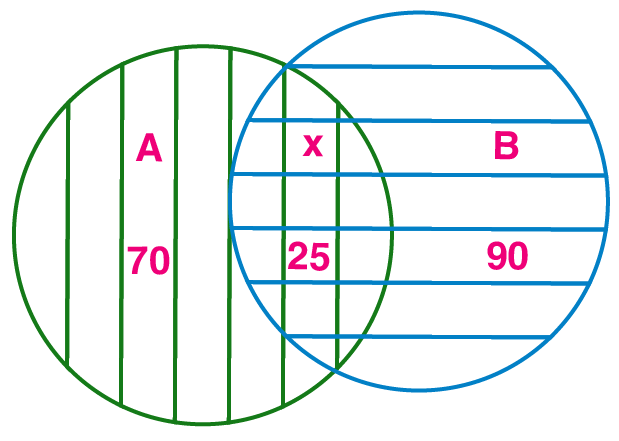As we know, n (A ∪ B) = n(A) + n(B) – n (A ∩ B)

⇒ n (A ∪ B) = 70 + 90 – 25

⇒ n (A ∪ B) = 160 – 25

⇒ n (A ∪ B) = 35

5. If n(A) = 20, n(B) = 28 and n (A ∪ B) = 36 then n (A ∩ B) =?

Solution:

Given n(A) = 20, n(B) = 28 and n (A ∪ B) = 36

As we know, n (A ∪ B) = n(A) + n(B) – n (A ∩ B)

⇒ n (A ∩ B) = 20 + 28 – 36

⇒ n (A ∩ B) = 48 – 36

⇒ n (A ∩ B)) = 12

6. In a class, 8 students out of 28 have a dog as their pet animal at home, 6 students have a cat as their pet animal. 10 students have dog and cat both, then how many students do not have a dog or cat as their pet animal at home?

Solution:

Given: n(A) = 8, n(B) = 6 and n (A ∩ B) = 10

n(A) = number of students having only dog as pet

n(B) = number of students having only cat as pet

n (A ∩ B) = number of trees planted by both of them together

Total students having a dog or cat as their pet animal at home = 8 + 6 + 10

= 24

Hence, number of students do not have a dog or cat as their pet animal at

Home = 28 – 24 = 4

7. Represent the union of two sets by Venn diagram for each of the following.

(i) A = {3, 4, 5, 7}
B = {1, 4, 8}

Solution:

Given A = {3, 4, 5, 7} and B = {1, 4, 8}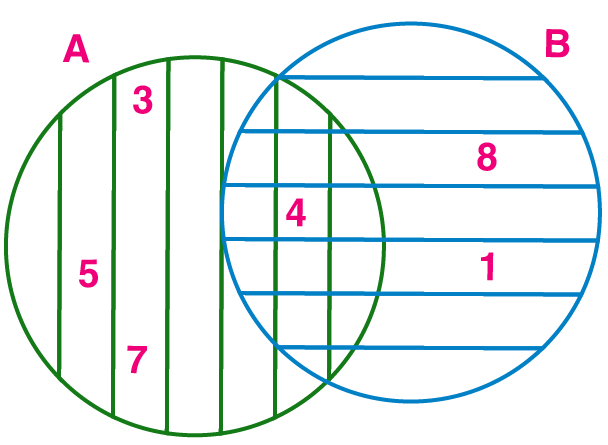(ii) P = {a, b, c, e, f}
Q = {I, m, n, e, b}

Solution:

Given P = {a, b, c, e, f}

Q = {I, m, n, e, b}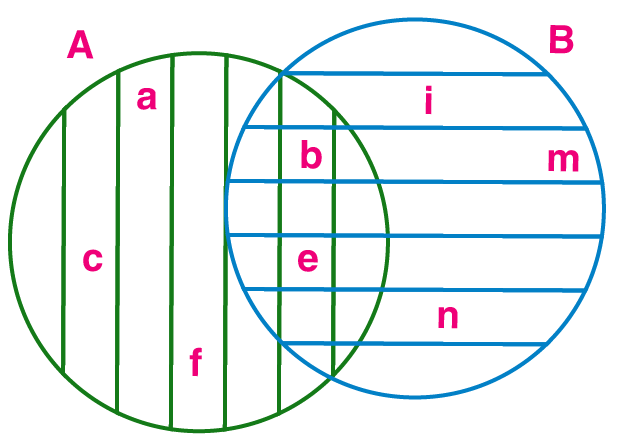(iii) X = {x/x is a prime number between 80 and 100}
Y = {y/y is an odd number between 90 and 100}

Solution:

Given X = {x/x is a prime number between 80 and 100}

Y = {y/y is an odd number between 90 and 100}

X = {83, 89, 93, 97}

Y = {91, 93, 95, 97, 99}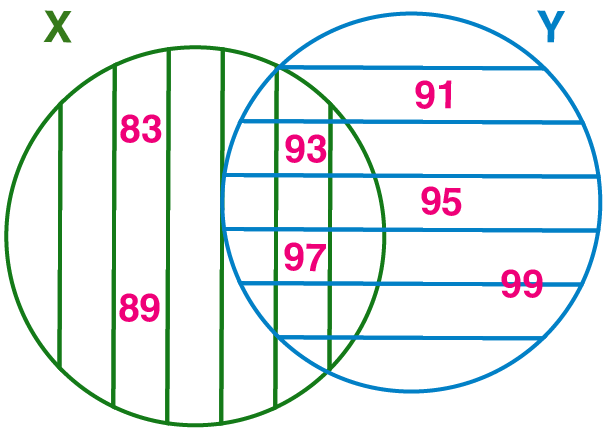8. Write the subset relations between the following sets.
X = set of all quadrilaterals.
Y = set of all rhombuses.
S = set of all squares.
T = set of all parallelograms.
V = set of all rectangles.

Solution:

Given X = set of all quadrilaterals.

Y = set of all rhombuses.

S = set of all squares.

T = set of all parallelograms.

V = set of all rectangles.

S ⊆ X, because all squares are quadrilaterals.

V ⊆ X, because all rectangles are quadrilaterals.

T ⊆ X, because all parallelograms are quadrilaterals.

S ⊆ Y, because all squares are rhombus.

S ⊆ V, because all squares are rectangles.

S ⊆ T, because all squares are parallelograms.

V ⊆ T, because all rectangles are parallelograms.

Y ⊆ T, because all rhombus are parallelograms.

9. If M is any set, then write M⋃ Φ and M ⋂ Φ

Solution:

Given: M is any set

As we know ϕ is null set.

Hence,

(M ∪ ϕ) = M

(M ∩ ϕ) = ϕ

10. Observe the Venn diagram and write the given sets U,A,B,A ∪ B and A ∩ B.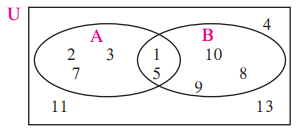Solution:

From the given Venn Diagram,

(i) U = {1, 2, 3, 4, 5, 7, 8, 9, 10, 11, 13}

(ii) A = {1, 2, 3, 5, 7}

(iii) B = {1, 5, 8, 9, 10}

(iv) (A ∪ B) = {1, 2, 3, 5, 7, 8, 9, 10}

(v) (A ∩ B) = {1, 5}

11. If n(A) = 7, n(B) = 13, n (A ∩ B) =4 then n (A ∪ B) =?

Solution:

Given n(A) = 7, n(B) = 13, n (A ∩ B) =4

As we know, n (A ∪ B) = n(A) + n(B) – n (A ∩ B)

⇒ n (A ∪ B) = 7 + 13 – 4

⇒ n (A ∪ B) = 20 – 4

⇒ n (A ∪ B) = 16

Sets are basically an organized collection of objects and can be either represented in roster form or set builder form. The objects that a set consists of are known as the elements of the set. Learn more about the concepts covered in the chapter Sets at BYJU’S.

## Frequently Asked Questions on Maharashtra State Board Solutions for Class 9 Maths Part 1 Chapter 1 Sets

### Is it possible to use this Maharashtra Board Class 9  Maths Solutions Chapter 1 to prepare for the exams?

Yes, it is highly recommended for the students to practise these solutions beforehand, as they form the basis for the questions that could get asked in the board exams.

### How can you use this Maharashtra Board Class 9 Maths Solutions Chapter 1 to prepare for the exams?

Students are advised to practise these questions first and then refer back to the solutions to analyse one’s performance and then rectify the mistakes so that they can avoid making any during the board exams.

### Is it possible to download Maharashtra Board Class 9 Maths Solutions Chapter 1?

Yes, we have provided the solutions as scrollable PDFs as well as we have given the clickable links for the students to access. Those who wish to can also check out the questions and the solutions from our webpage.